# 《利用Python进行数据分析·第2版》第9章 绘图和可视化

+关注继续查看

matplotlib是一个用于创建出版质量图表的桌面绘图包（主要是2D方面）。该项目是由John Hunter于2002年启动的，其目的是为Python构建一个MATLAB式的绘图接口。matplotlib和IPython社区进行合作，简化了从IPython shell（包括现在的Jupyter notebook）进行交互式绘图。matplotlib支持各种操作系统上许多不同的GUI后端，而且还能将图片导出为各种常见的矢量（vector）和光栅（raster）图：PDF、SVG、JPG、PNG、BMP、GIF等。除了几张，本书中的大部分图都是用它生成的。

%matplotlib notebook


# 9.1 matplotlib API入门

matplotlib的通常引入约定是：

In : import matplotlib.pyplot as plt


In : import numpy as np

In : data = np.arange(10)

In : data
Out: array([0, 1, 2, 3, 4, 5, 6, 7, 8, 9])

In : plt.plot(data)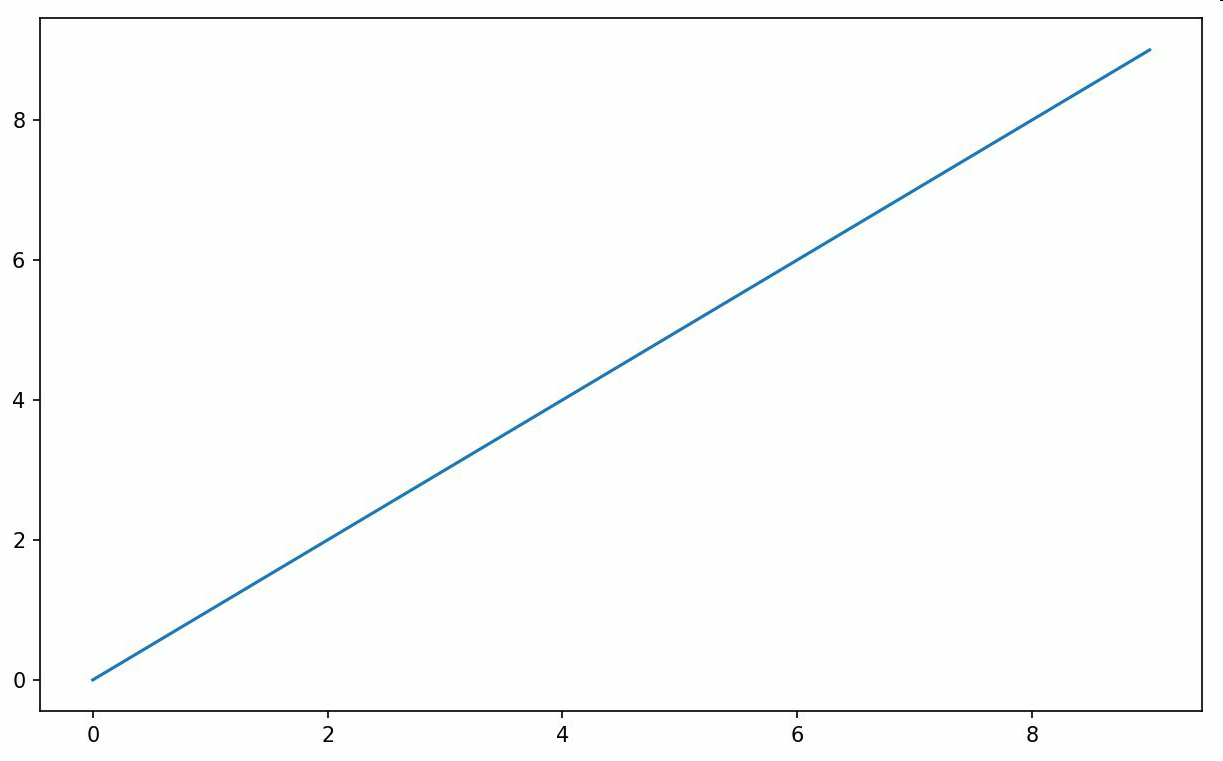## Figure和Subplot

matplotlib的图像都位于Figure对象中。你可以用plt.figure创建一个新的Figure：

In : fig = plt.figure()


In : ax1 = fig.add_subplot(2, 2, 1)


In : ax2 = fig.add_subplot(2, 2, 2)

In : ax3 = fig.add_subplot(2, 2, 3)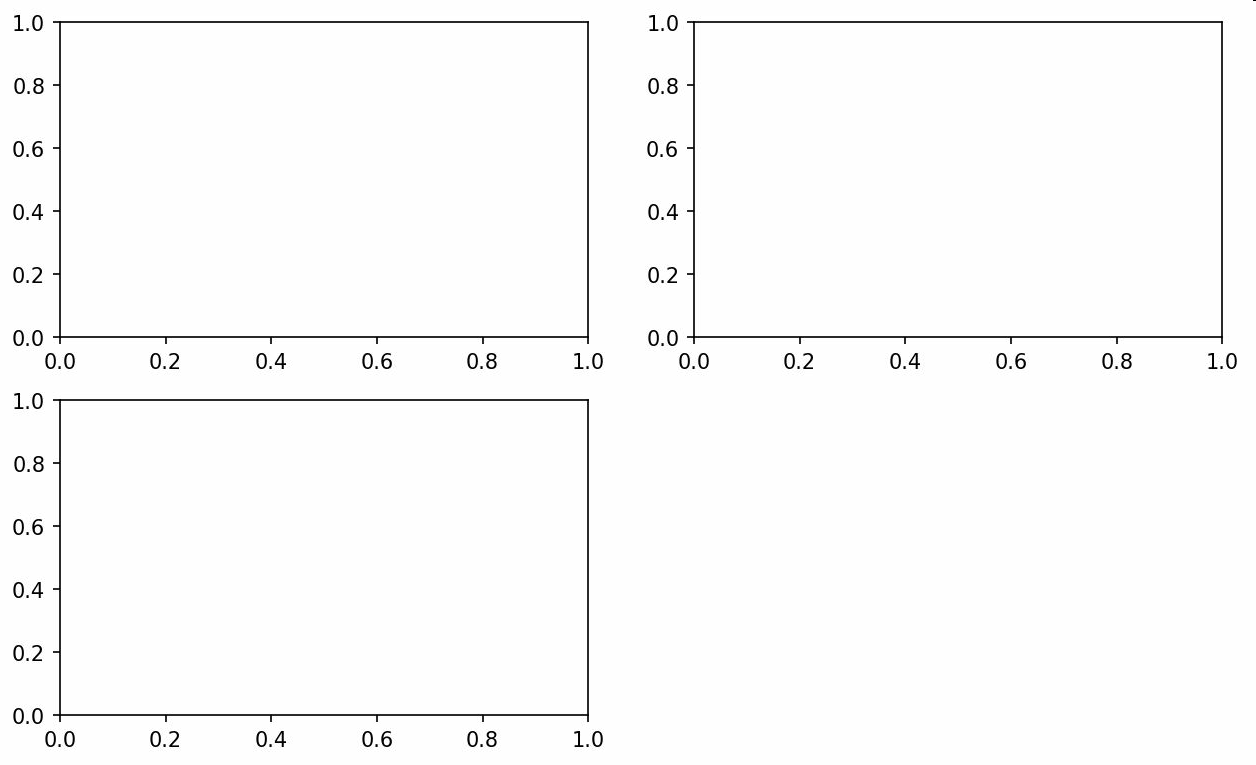fig = plt.figure()


In : plt.plot(np.random.randn(50).cumsum(), 'k--')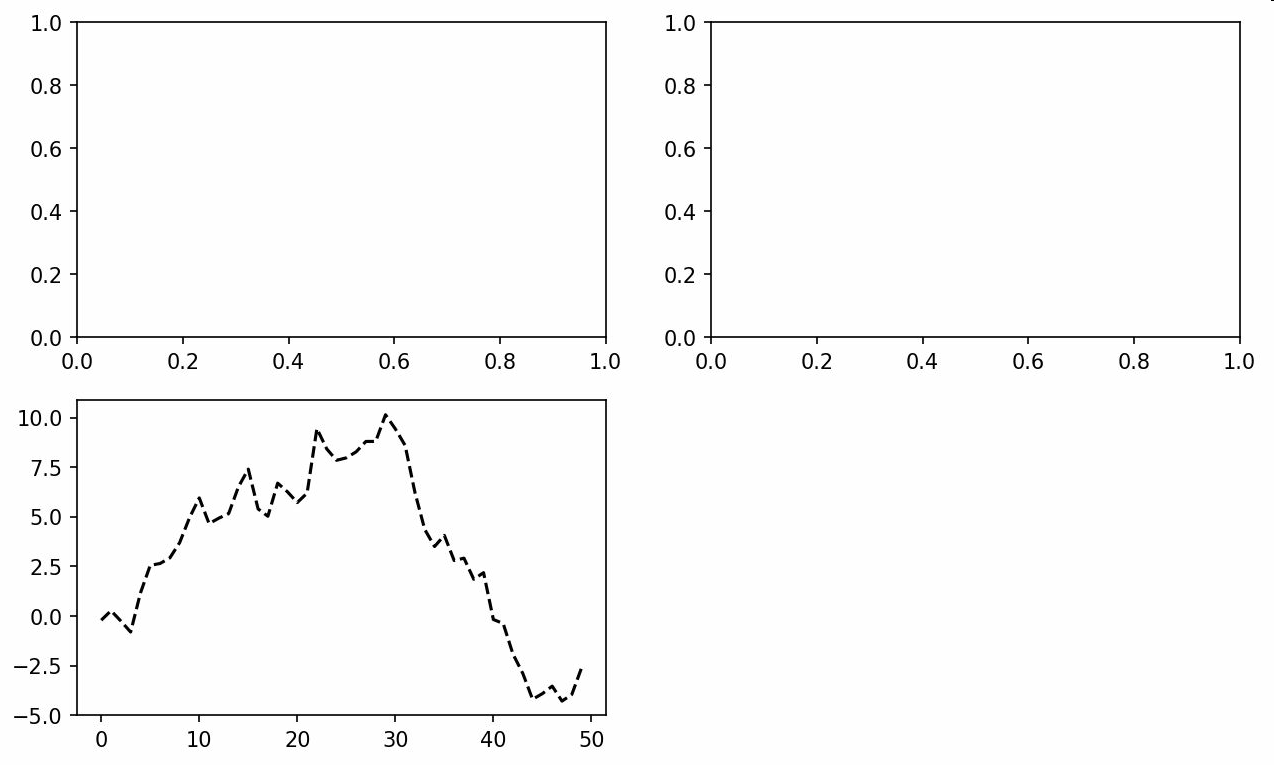In : ax1.hist(np.random.randn(100), bins=20, color='k', alpha=0.3)

In : ax2.scatter(np.arange(30), np.arange(30) + 3 * np.random.randn(30))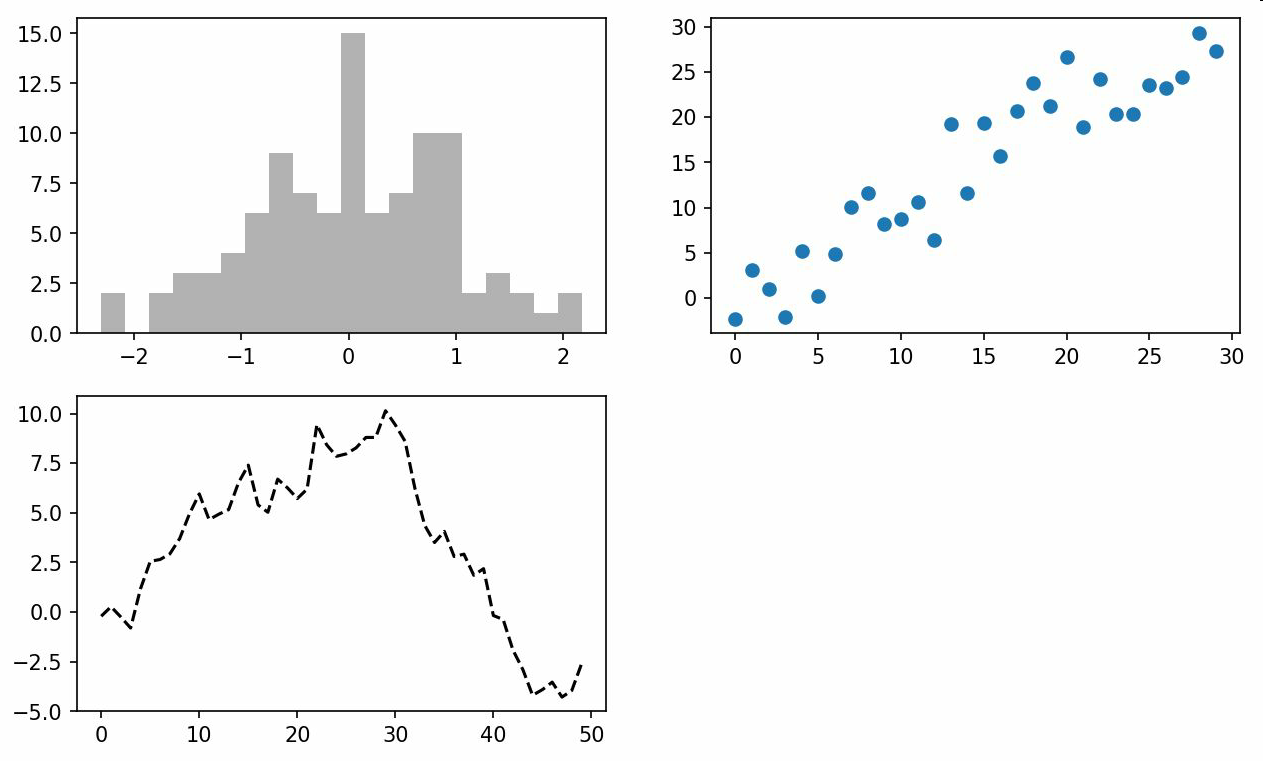In : fig, axes = plt.subplots(2, 3)

In : axes
Out:
array([[<matplotlib.axes._subplots.AxesSubplot object at 0x7fb626374048>,
<matplotlib.axes._subplots.AxesSubplot object at 0x7fb62625db00>,
<matplotlib.axes._subplots.AxesSubplot object at 0x7fb6262f6c88>],
[<matplotlib.axes._subplots.AxesSubplot object at 0x7fb6261a36a0>,
<matplotlib.axes._subplots.AxesSubplot object at 0x7fb626181860>,
<matplotlib.axes._subplots.AxesSubplot object at 0x7fb6260fd4e0>]], dtype
=object)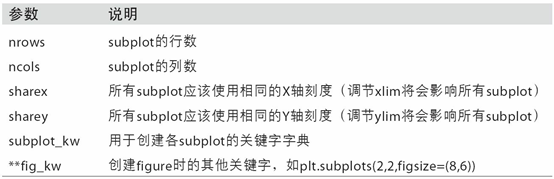## 调整subplot周围的间距

subplots_adjust(left=None, bottom=None, right=None, top=None,
wspace=None, hspace=None)


wspace和hspace用于控制宽度和高度的百分比，可以用作subplot之间的间距。下面是一个简单的例子，其中我将间距收缩到了0（如图9-5所示）：

fig, axes = plt.subplots(2, 2, sharex=True, sharey=True)
for i in range(2):
for j in range(2):
axes[i, j].hist(np.random.randn(500), bins=50, color='k', alpha=0.5)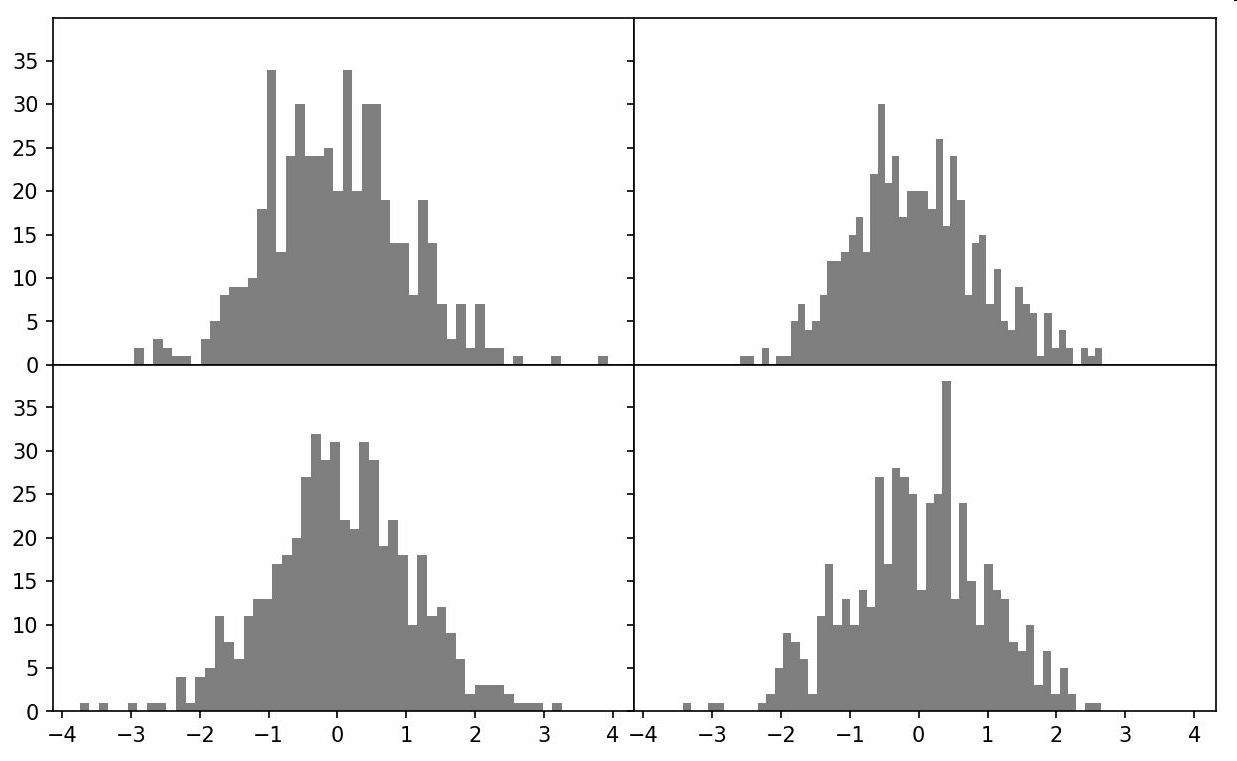## 颜色、标记和线型

matplotlib的plot函数接受一组X和Y坐标，还可以接受一个表示颜色和线型的字符串缩写。例如，要根据x和y绘制绿色虚线，你可以执行如下代码：

ax.plot(x, y, 'g--')


ax.plot(x, y, linestyle='--', color='g')


In : from numpy.random import randn

In : plt.plot(randn(30).cumsum(), 'ko--')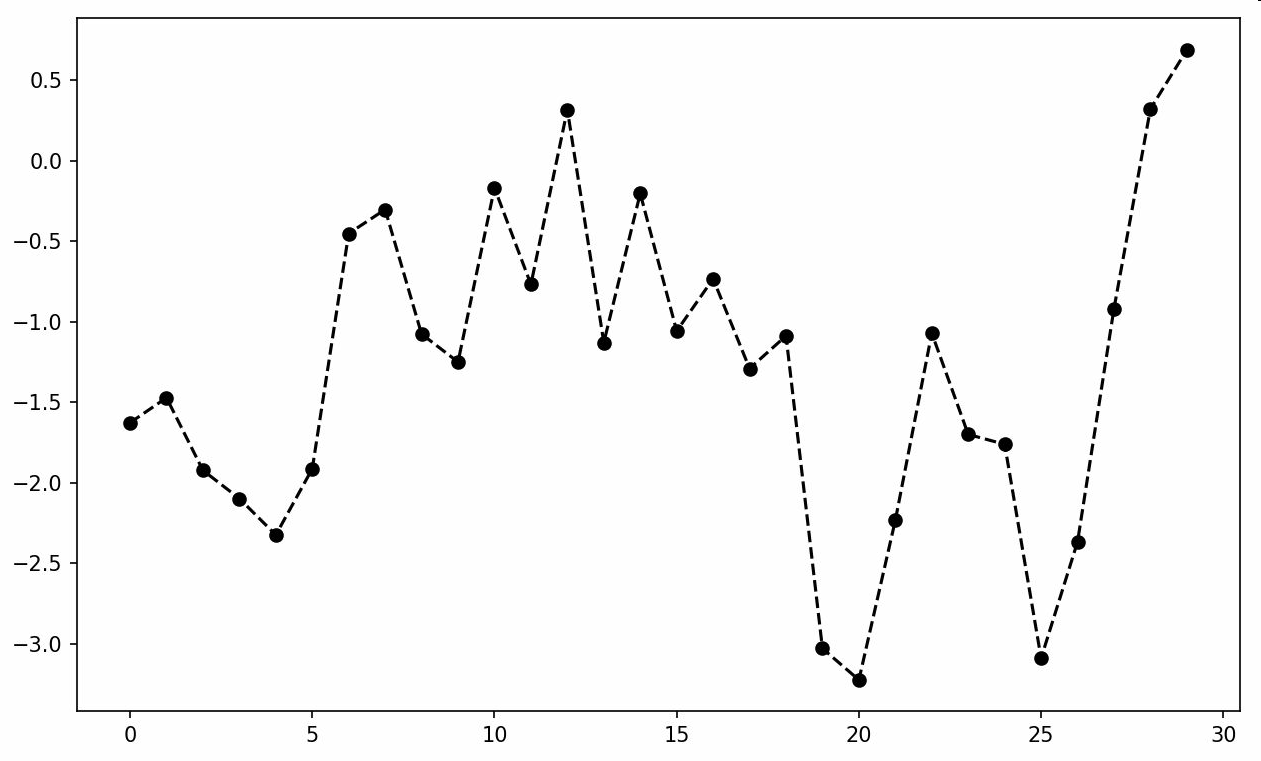plot(randn(30).cumsum(), color='k', linestyle='dashed', marker='o')


In : data = np.random.randn(30).cumsum()

In : plt.plot(data, 'k--', label='Default')
Out: [<matplotlib.lines.Line2D at 0x7fb624d86160>]

In : plt.plot(data, 'k-', drawstyle='steps-post', label='steps-post')
Out: [<matplotlib.lines.Line2D at 0x7fb624d869e8>]

In : plt.legend(loc='best')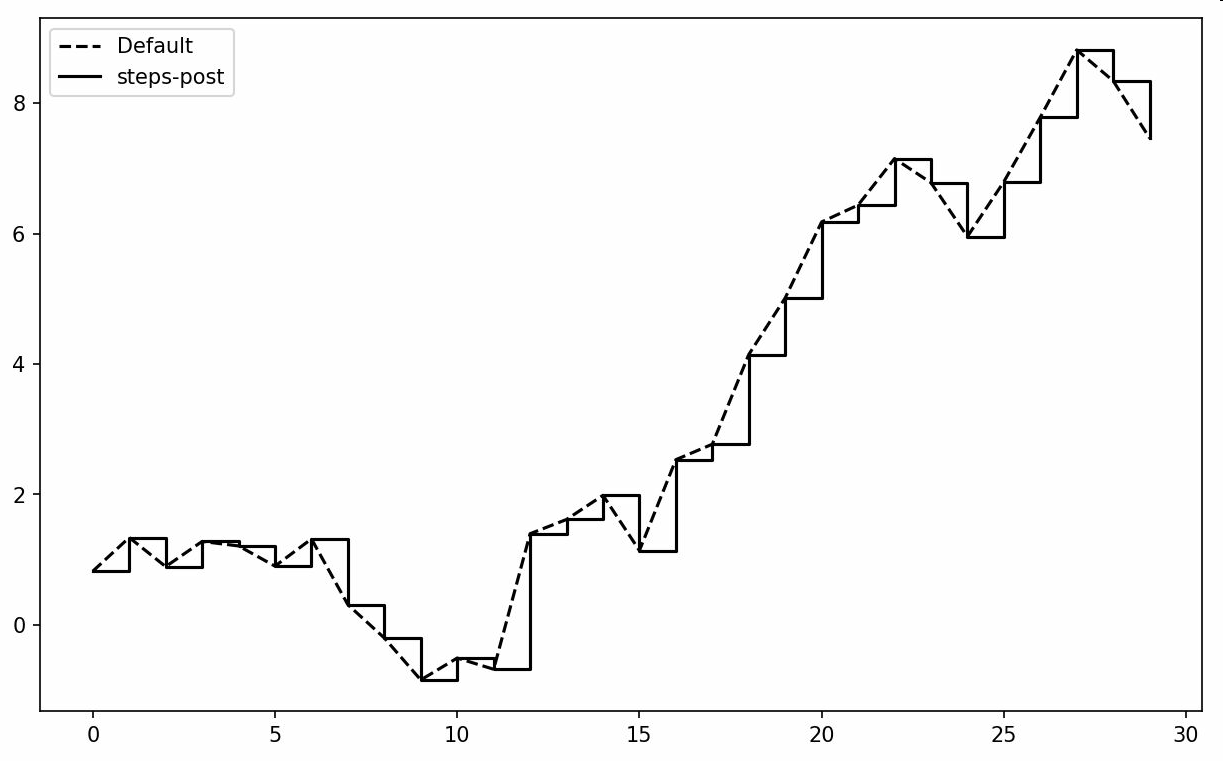## 刻度、标签和图例

pyplot接口的设计目的就是交互式使用，含有诸如xlim、xticks和xticklabels之类的方法。它们分别控制图表的范围、刻度位置、刻度标签等。其使用方式有以下两种：

• 调用时不带参数，则返回当前的参数值（例如，plt.xlim()返回当前的X轴绘图范围）。
• 调用时带参数，则设置参数值（例如，plt.xlim([0,10])会将X轴的范围设置为0到10）。

## 设置标题、轴标签、刻度以及刻度标签

In : fig = plt.figure()

In : ax = fig.add_subplot(1, 1, 1)

In : ax.plot(np.random.randn(1000).cumsum())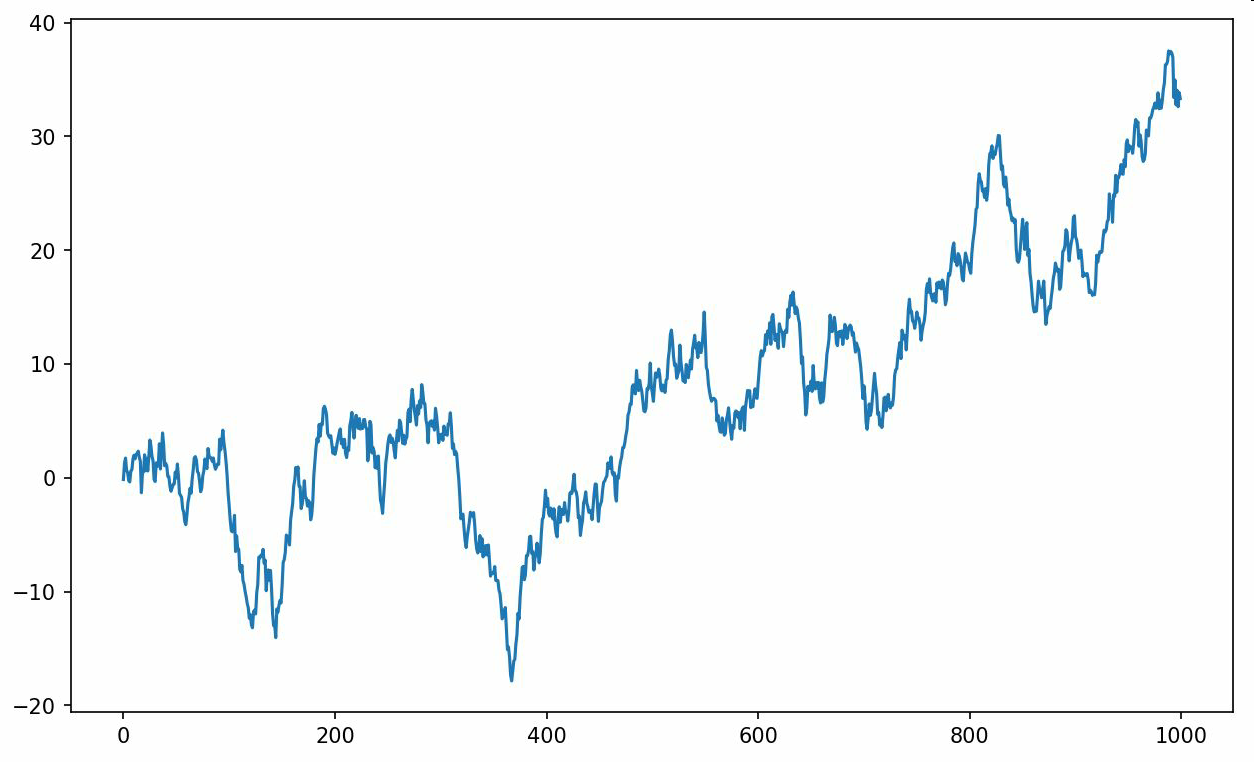In : ticks = ax.set_xticks([0, 250, 500, 750, 1000])

In : labels = ax.set_xticklabels(['one', 'two', 'three', 'four', 'five'],
....:                             rotation=30, fontsize='small')


rotation选项设定x刻度标签倾斜30度。最后，再用set_xlabel为X轴设置一个名称，并用set_title设置一个标题（见图9-9的结果）：

In : ax.set_title('My first matplotlib plot')
Out: <matplotlib.text.Text at 0x7fb624d055f8>

In : ax.set_xlabel('Stages')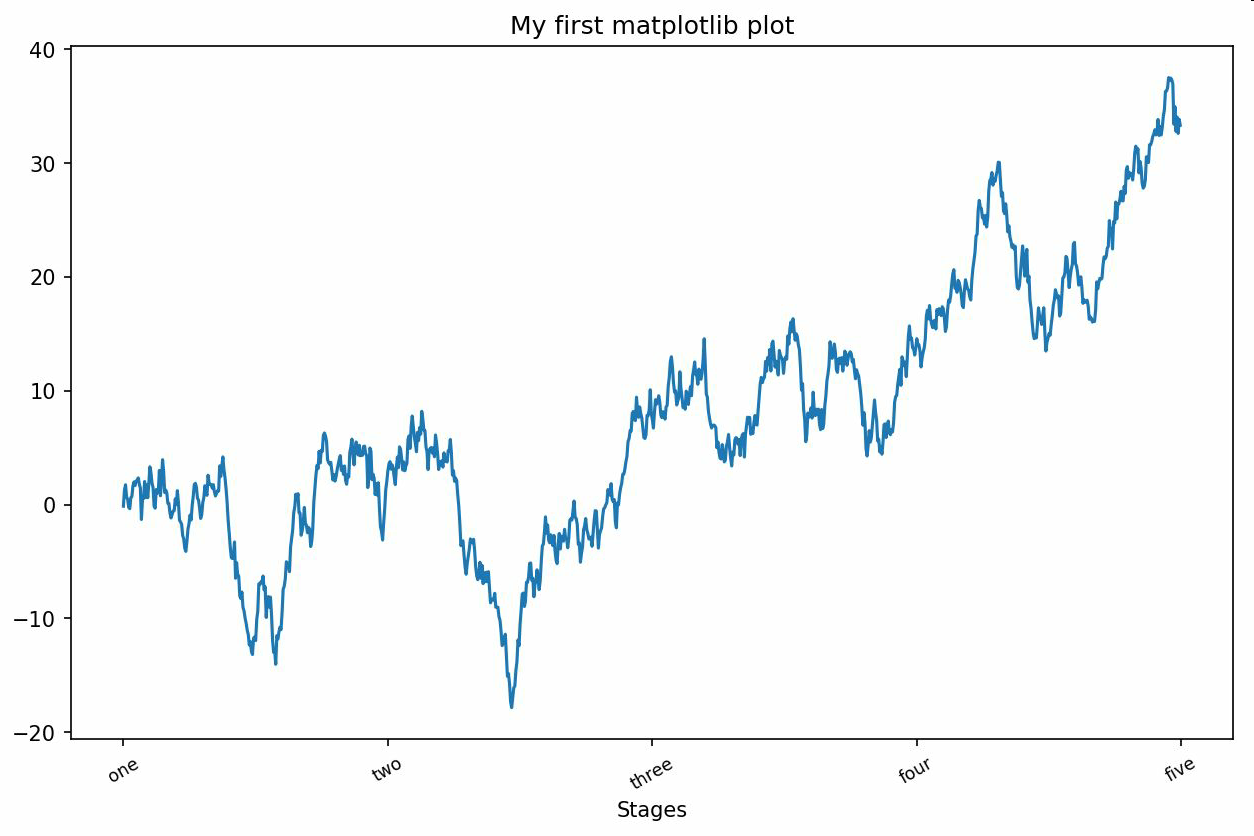Y轴的修改方式与此类似，只需将上述代码中的x替换为y即可。轴的类有集合方法，可以批量设定绘图选项。前面的例子，也可以写为：

props = {
'title': 'My first matplotlib plot',
'xlabel': 'Stages'
}
ax.set(**props)


## 添加图例

In : from numpy.random import randn

In : fig = plt.figure(); ax = fig.add_subplot(1, 1, 1)

In : ax.plot(randn(1000).cumsum(), 'k', label='one')
Out: [<matplotlib.lines.Line2D at 0x7fb624bdf860>]

In : ax.plot(randn(1000).cumsum(), 'k--', label='two')
Out: [<matplotlib.lines.Line2D at 0x7fb624be90f0>]

In : ax.plot(randn(1000).cumsum(), 'k.', label='three')
Out: [<matplotlib.lines.Line2D at 0x7fb624be9160>]


In : ax.legend(loc='best')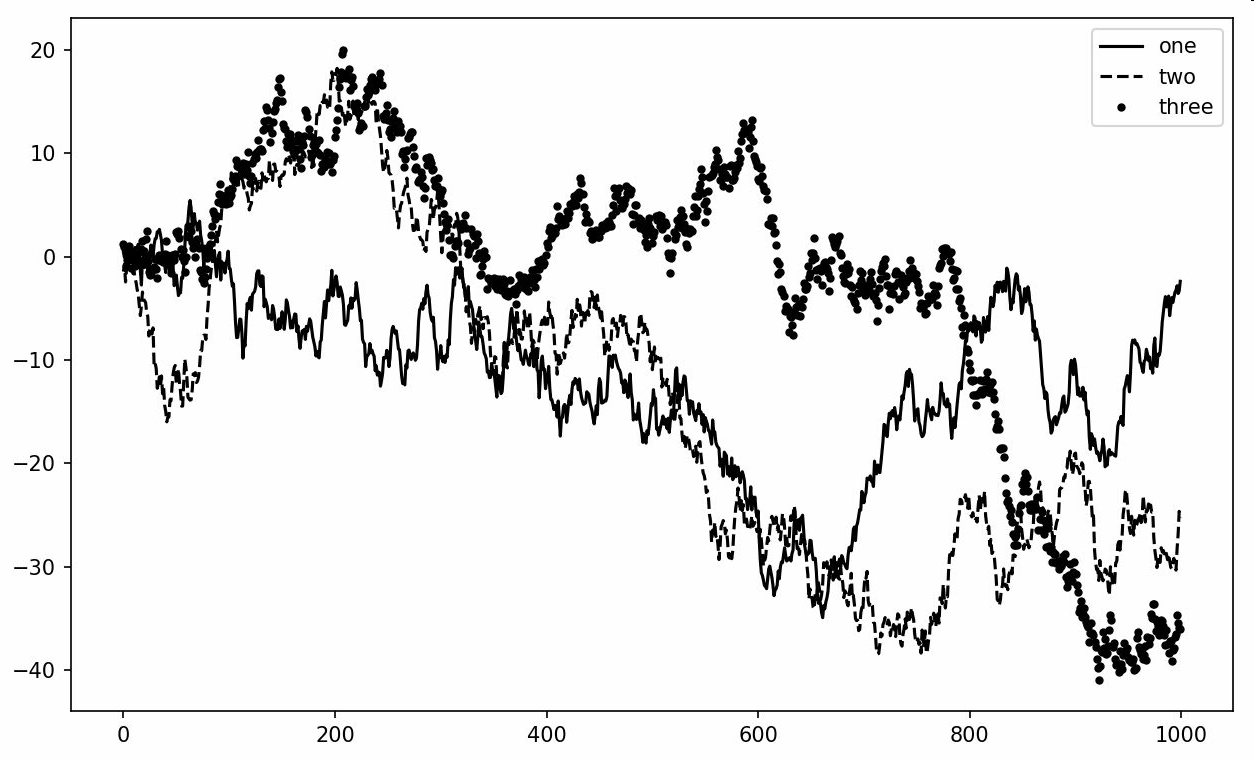legend方法有几个其它的loc位置参数选项。请查看文档字符串（使用ax.legend?）。

loc告诉matplotlib要将图例放在哪。如果你不是吹毛求疵的话，"best"是不错的选择，因为它会选择最不碍事的位置。要从图例中去除一个或多个元素，不传入label或传入label='nolegend'即可。（中文第一版这里把best错写成了beat）

## 注解以及在Subplot上绘图

ax.text(x, y, 'Hello world!',
family='monospace', fontsize=10)


from datetime import datetime

fig = plt.figure()

spx = data['SPX']

spx.plot(ax=ax, style='k-')

crisis_data = [
(datetime(2007, 10, 11), 'Peak of bull market'),
(datetime(2008, 3, 12), 'Bear Stearns Fails'),
(datetime(2008, 9, 15), 'Lehman Bankruptcy')
]

for date, label in crisis_data:
ax.annotate(label, xy=(date, spx.asof(date) + 75),
xytext=(date, spx.asof(date) + 225),
horizontalalignment='left', verticalalignment='top')

# Zoom in on 2007-2010
ax.set_xlim(['1/1/2007', '1/1/2011'])
ax.set_ylim([600, 1800])

ax.set_title('Important dates in the 2008-2009 financial crisis')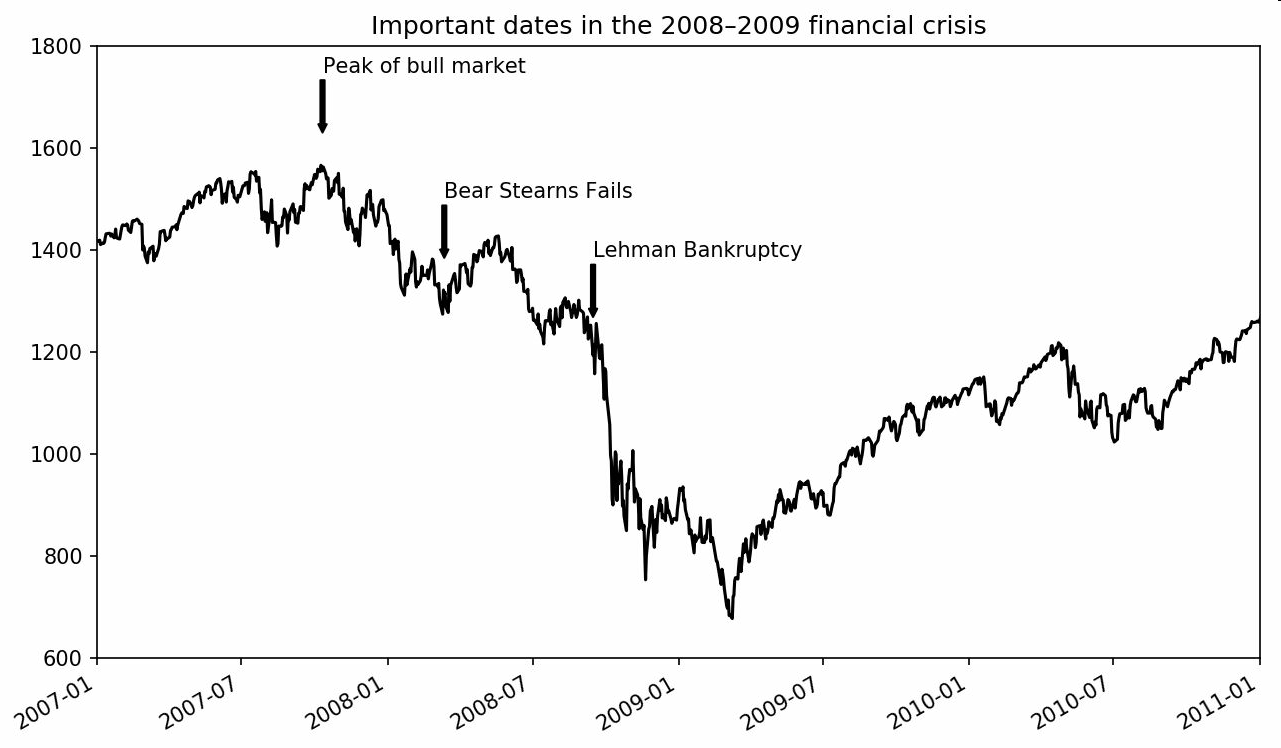fig = plt.figure()

rect = plt.Rectangle((0.2, 0.75), 0.4, 0.15, color='k', alpha=0.3)
circ = plt.Circle((0.7, 0.2), 0.15, color='b', alpha=0.3)
pgon = plt.Polygon([[0.15, 0.15], [0.35, 0.4], [0.2, 0.6]],
color='g', alpha=0.5)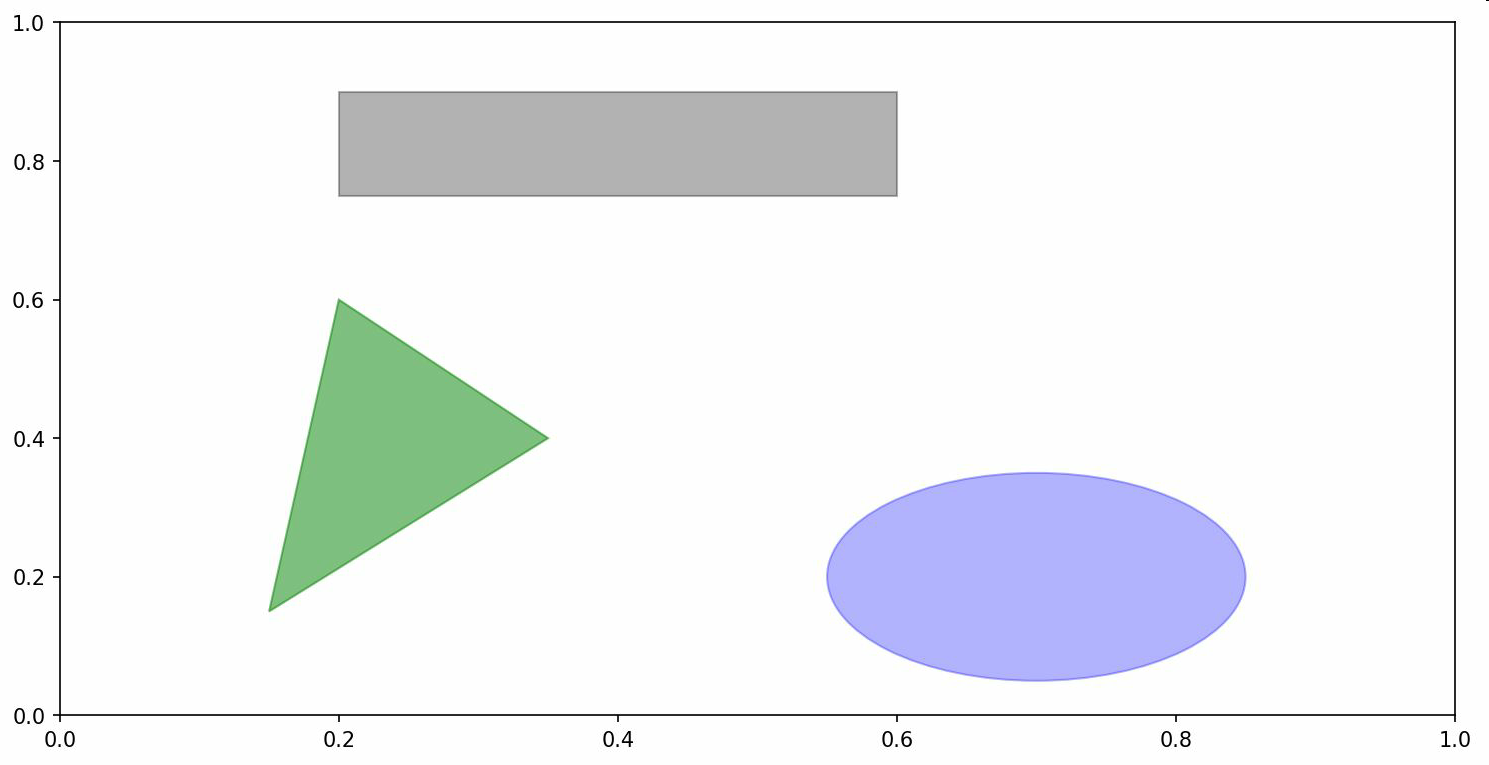## 将图表保存到文件

plt.savefig('figpath.svg')


plt.savefig('figpath.png', dpi=400, bbox_inches='tight')


savefig并非一定要写入磁盘，也可以写入任何文件型的对象，比如BytesIO：

from io import BytesIO
buffer = BytesIO()
plt.savefig(buffer)
plot_data = buffer.getvalue()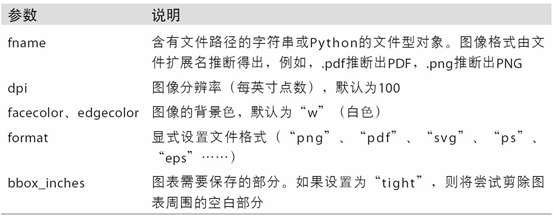## matplotlib配置

matplotlib自带一些配色方案，以及为生成出版质量的图片而设定的默认配置信息。幸运的是，几乎所有默认行为都能通过一组全局参数进行自定义，它们可以管理图像大小、subplot边距、配色方案、字体大小、网格类型等。一种Python编程方式配置系统的方法是使用rc方法。例如，要将全局的图像默认大小设置为10×10，你可以执行：

plt.rc('figure', figsize=(10, 10))


rc的第一个参数是希望自定义的对象，如'figure'、'axes'、'xtick'、'ytick'、'grid'、'legend'等。其后可以跟上一系列的关键字参数。一个简单的办法是将这些选项写成一个字典：

font_options = {'family' : 'monospace',
'weight' : 'bold',
'size'   : 'small'}
plt.rc('font', **font_options)


# 9.2 使用pandas和seaborn绘图

matplotlib实际上是一种比较低级的工具。要绘制一张图表，你组装一些基本组件就行：数据展示（即图表类型：线型图、柱状图、盒形图、散布图、等值线图等）、图例、标题、刻度标签以及其他注解型信息。

## 线型图

Series和DataFrame都有一个用于生成各类图表的plot方法。默认情况下，它们所生成的是线型图（如图9-13所示）：

In : s = pd.Series(np.random.randn(10).cumsum(), index=np.arange(0, 100, 10))

In : s.plot()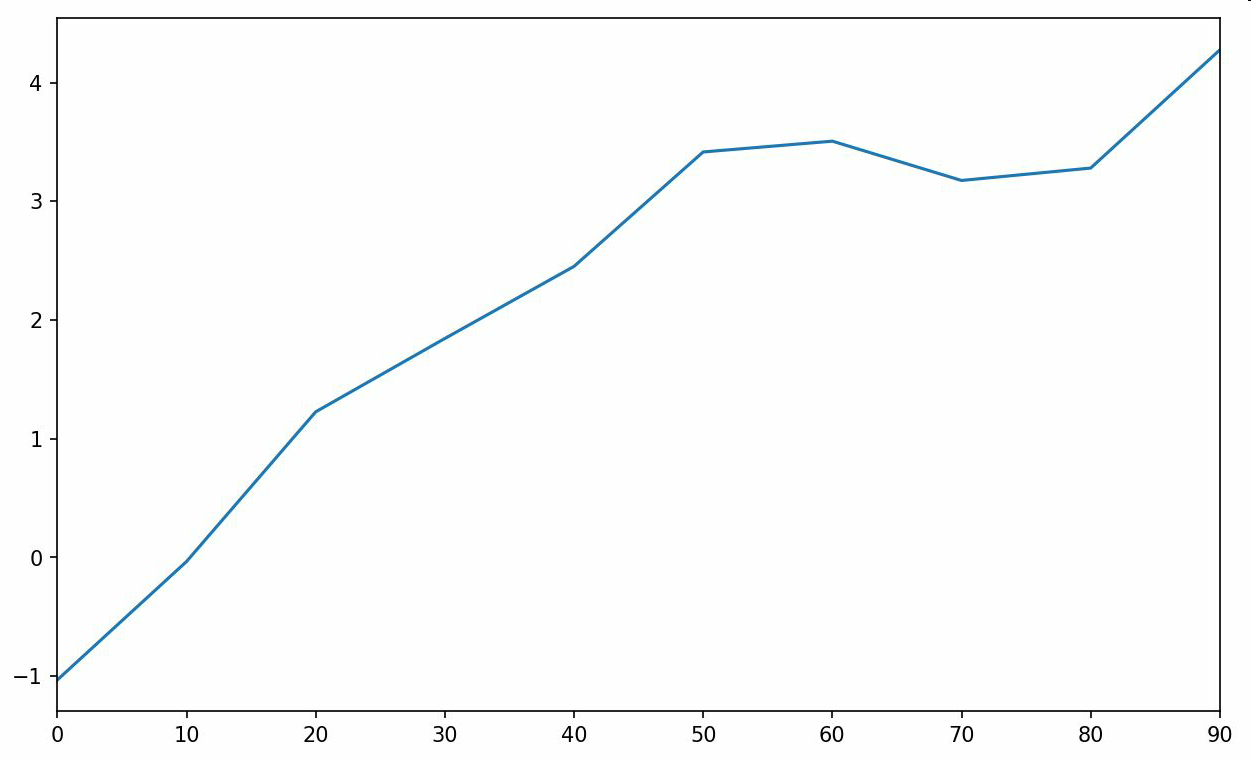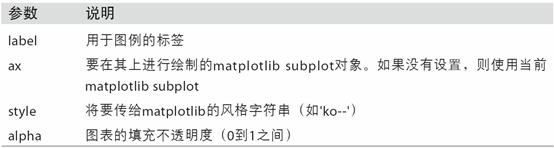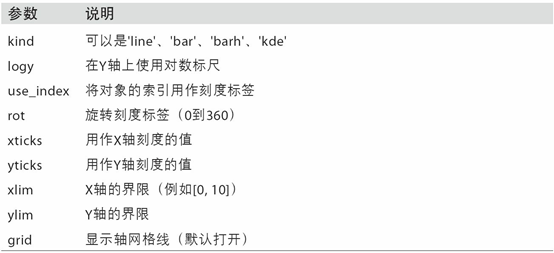pandas的大部分绘图方法都有一个可选的ax参数，它可以是一个matplotlib的subplot对象。这使你能够在网格布局中更为灵活地处理subplot的位置。

DataFrame的plot方法会在一个subplot中为各列绘制一条线，并自动创建图例（如图9-14所示）：

In : df = pd.DataFrame(np.random.randn(10, 4).cumsum(0),
....:                   columns=['A', 'B', 'C', 'D'],
....:                   index=np.arange(0, 100, 10))

In : df.plot()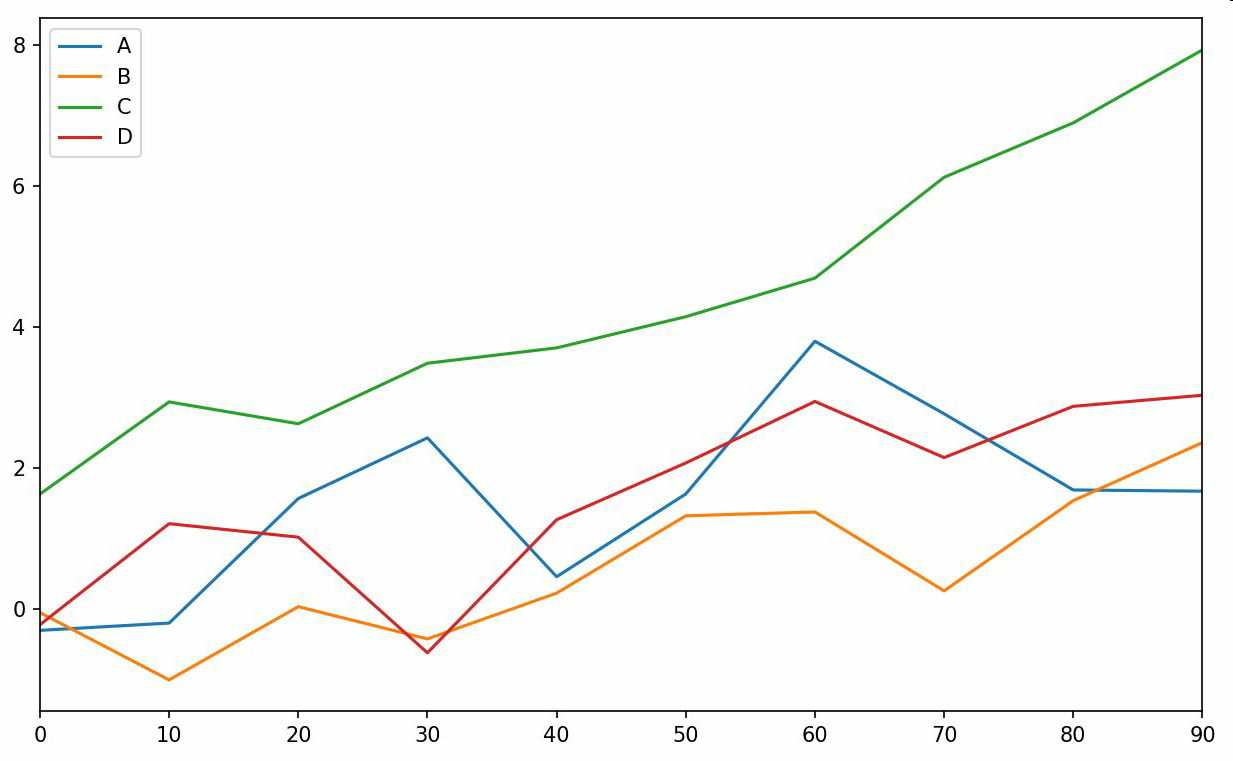plot属性包含一批不同绘图类型的方法。例如，df.plot()等价于df.plot.line()。后面会学习这些方法。

DataFrame还有一些用于对列进行灵活处理的选项，例如，是要将所有列都绘制到一个subplot中还是创建各自的subplot。详细信息请参见表9-4。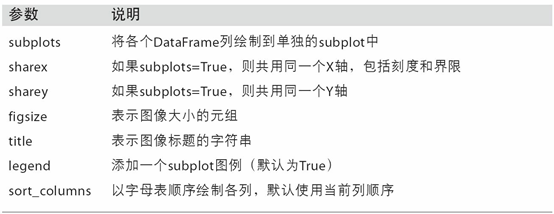## 柱状图

plot.bar()和plot.barh()分别绘制水平和垂直的柱状图。这时，Series和DataFrame的索引将会被用作X（bar）或Y（barh）刻度（如图9-15所示）：

In : fig, axes = plt.subplots(2, 1)

In : data = pd.Series(np.random.rand(16), index=list('abcdefghijklmnop'))

In : data.plot.bar(ax=axes, color='k', alpha=0.7)
Out: <matplotlib.axes._subplots.AxesSubplot at 0x7fb62493d470>

In : data.plot.barh(ax=axes, color='k', alpha=0.7)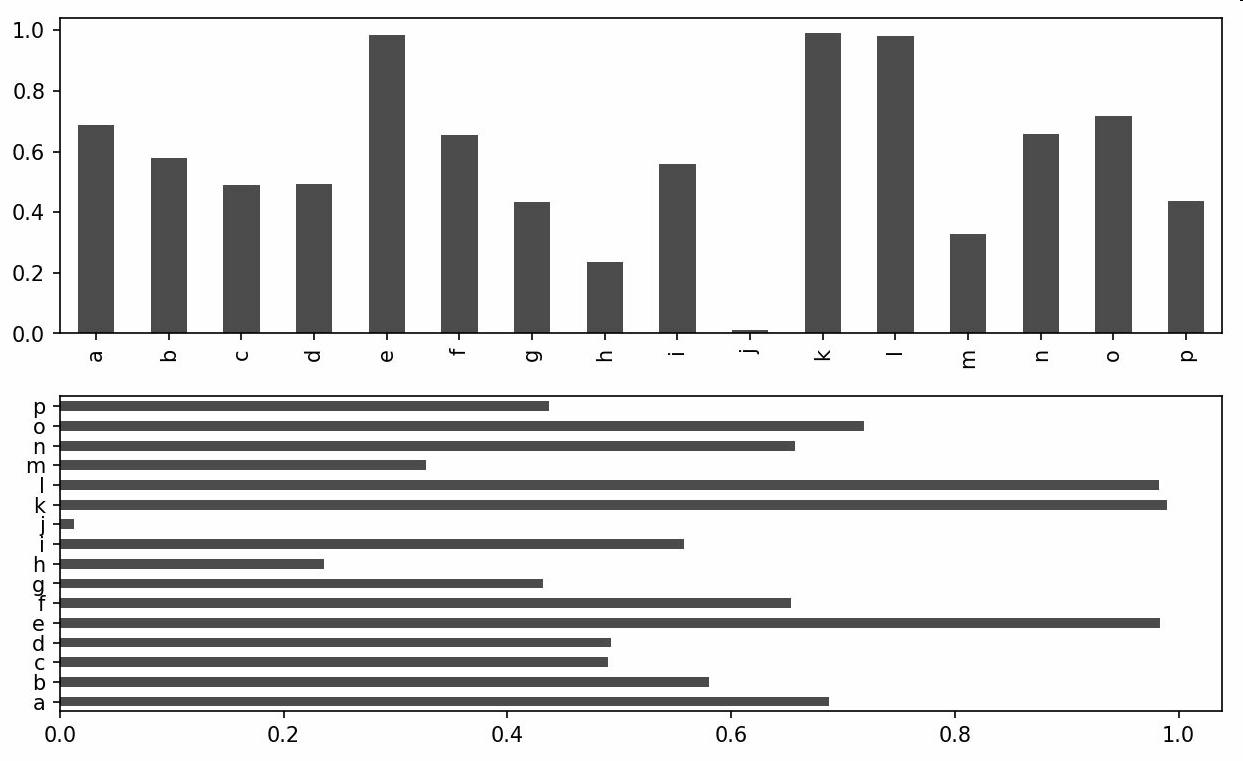color='k'和alpha=0.7设定了图形的颜色为黑色，并使用部分的填充透明度。对于DataFrame，柱状图会将每一行的值分为一组，并排显示，如图9-16所示：

In : df = pd.DataFrame(np.random.rand(6, 4),
....:                   index=['one', 'two', 'three', 'four', 'five', 'six'],
....:                   columns=pd.Index(['A', 'B', 'C', 'D'], name='Genus'))

In : df
Out:
Genus         A         B         C         D
one    0.370670  0.602792  0.229159  0.486744
two    0.420082  0.571653  0.049024  0.880592
three  0.814568  0.277160  0.880316  0.431326
four   0.374020  0.899420  0.460304  0.100843
five   0.433270  0.125107  0.494675  0.961825
six    0.601648  0.478576  0.205690  0.560547

In : df.plot.bar()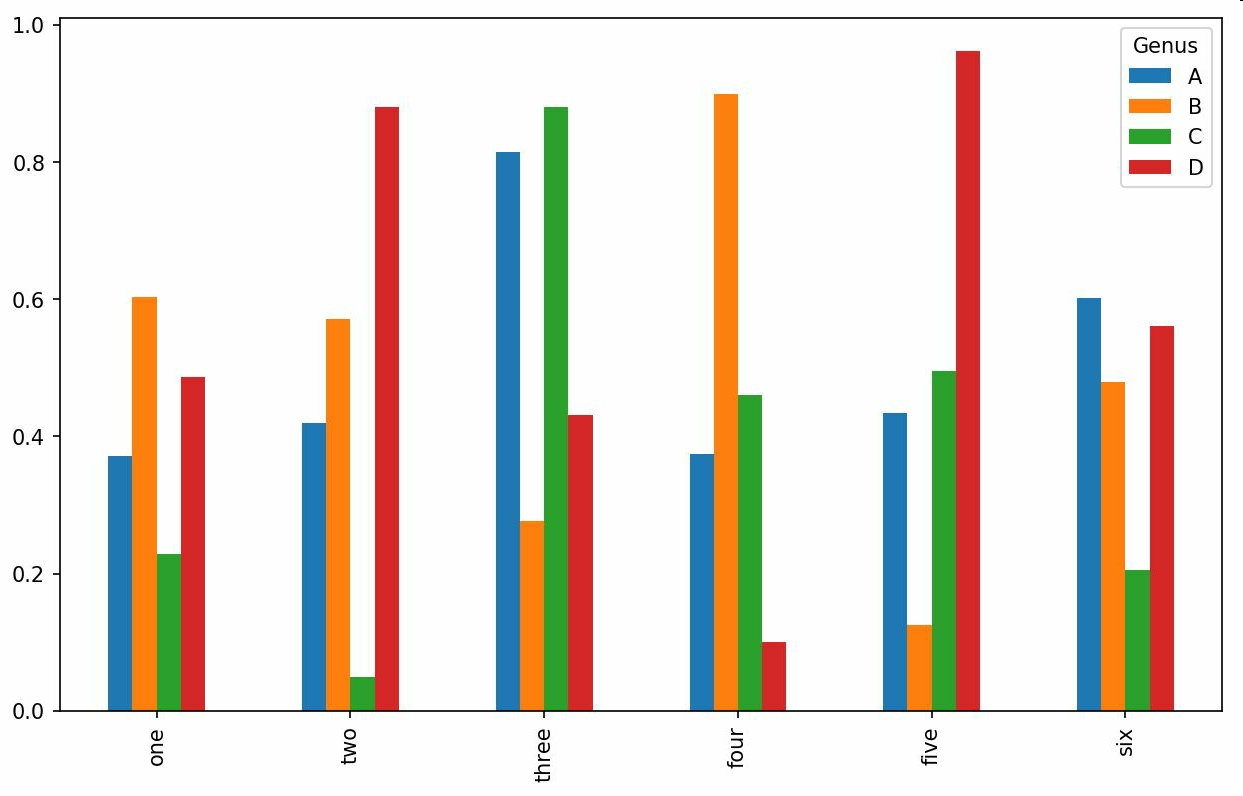In : df.plot.barh(stacked=True, alpha=0.5)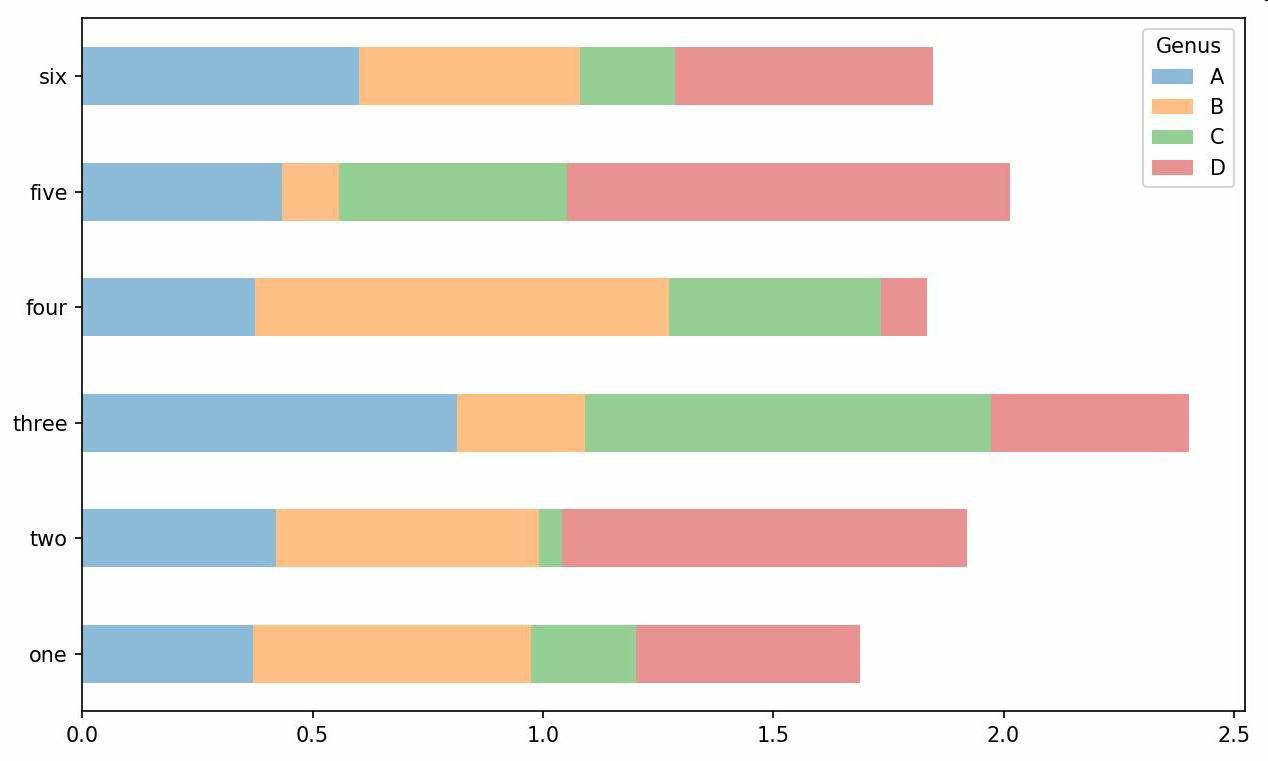In : tips = pd.read_csv('examples/tips.csv')

In : party_counts = pd.crosstab(tips['day'], tips['size'])

In : party_counts
Out:
size  1   2   3   4  5  6
day
Fri   1  16   1   1  0  0
Sat   2  53  18  13  1  0
Sun   0  39  15  18  3  1
Thur  1  48   4   5  1  3

# Not many 1- and 6-person parties
In : party_counts = party_counts.loc[:, 2:5]


# Normalize to sum to 1
In : party_pcts = party_counts.div(party_counts.sum(1), axis=0)

In : party_pcts
Out:
size         2         3         4         5
day
Fri   0.888889  0.055556  0.055556  0.000000
Sat   0.623529  0.211765  0.152941  0.011765
Sun   0.520000  0.200000  0.240000  0.040000
Thur  0.827586  0.068966  0.086207  0.017241

In : party_pcts.plot.bar()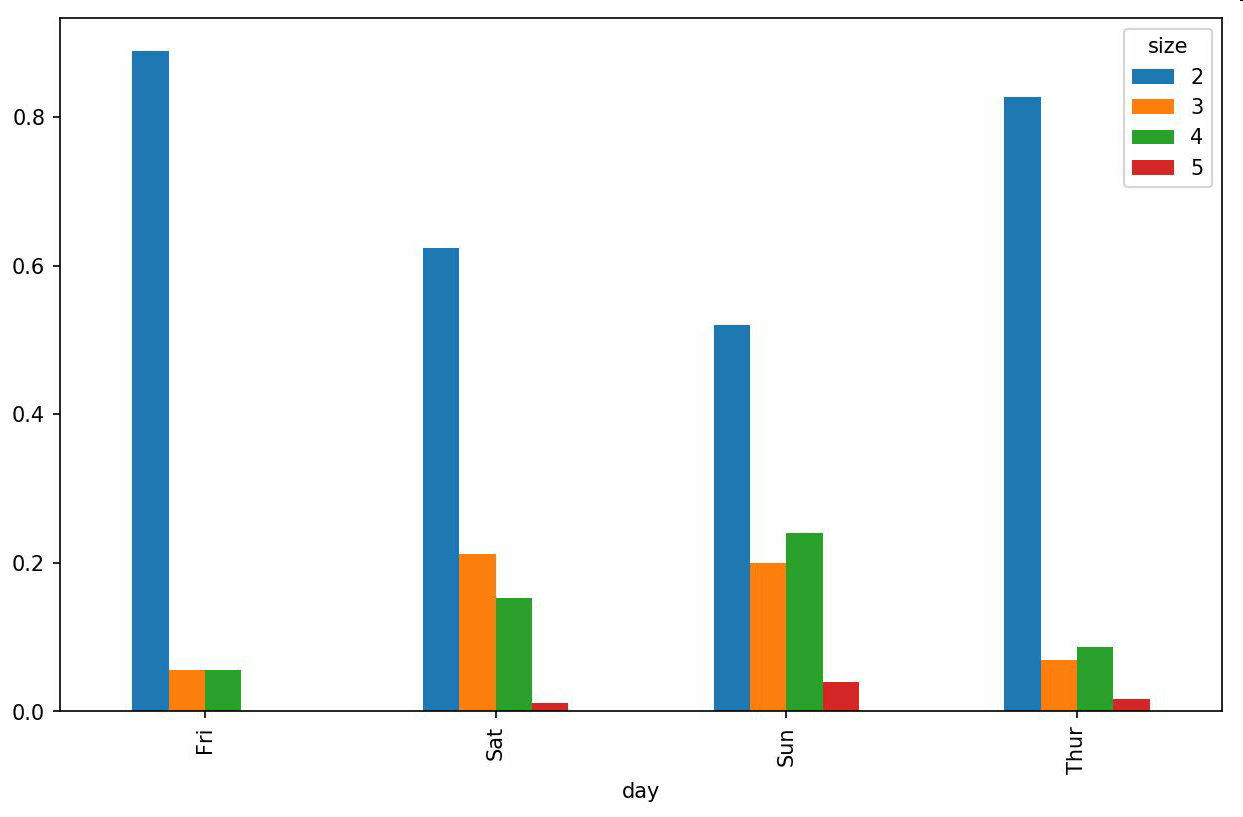In : import seaborn as sns

In : tips['tip_pct'] = tips['tip'] / (tips['total_bill'] - tips['tip'])

Out:
total_bill   tip smoker  day    time  size   tip_pct
0       16.99  1.01     No  Sun  Dinner     2  0.063204
1       10.34  1.66     No  Sun  Dinner     3  0.191244
2       21.01  3.50     No  Sun  Dinner     3  0.199886
3       23.68  3.31     No  Sun  Dinner     2  0.162494
4       24.59  3.61     No  Sun  Dinner     4  0.172069

In : sns.barplot(x='tip_pct', y='day', data=tips, orient='h')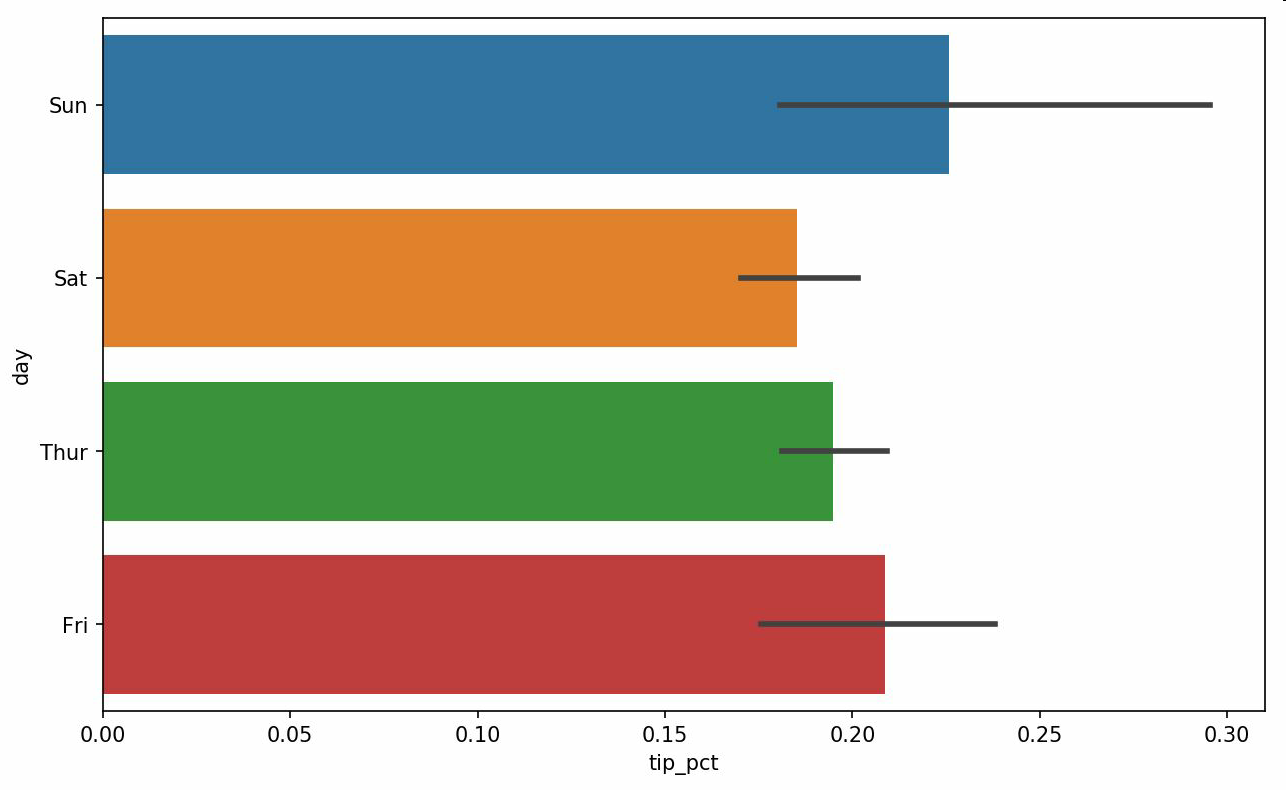seaborn的绘制函数使用data参数，它可能是pandas的DataFrame。其它的参数是关于列的名字。因为一天的每个值有多次观察，柱状图的值是tip_pct的平均值。绘制在柱状图上的黑线代表95%置信区间（可以通过可选参数配置）。

seaborn.barplot有颜色选项，使我们能够通过一个额外的值设置（见图9-20）：

In : sns.barplot(x='tip_pct', y='day', hue='time', data=tips, orient='h')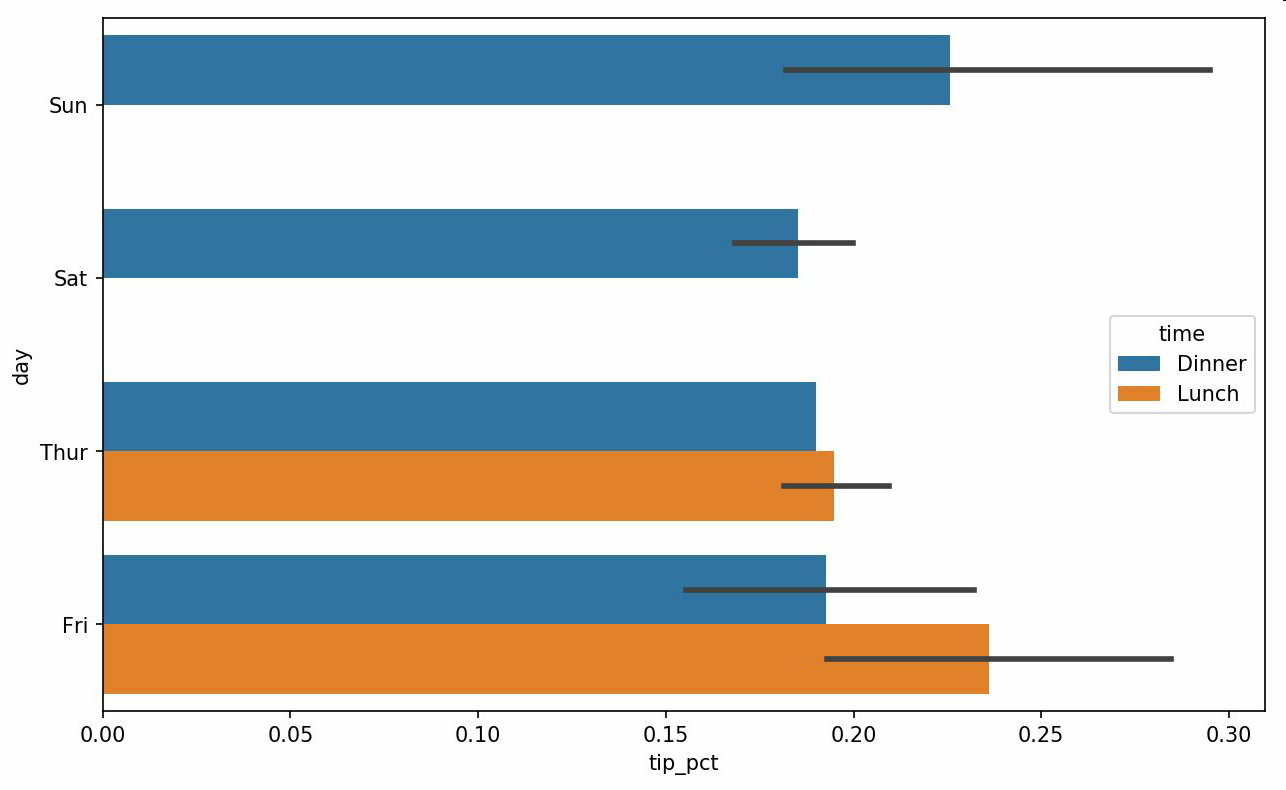In : sns.set(style="whitegrid")


## 直方图和密度图

In : tips['tip_pct'].plot.hist(bins=50)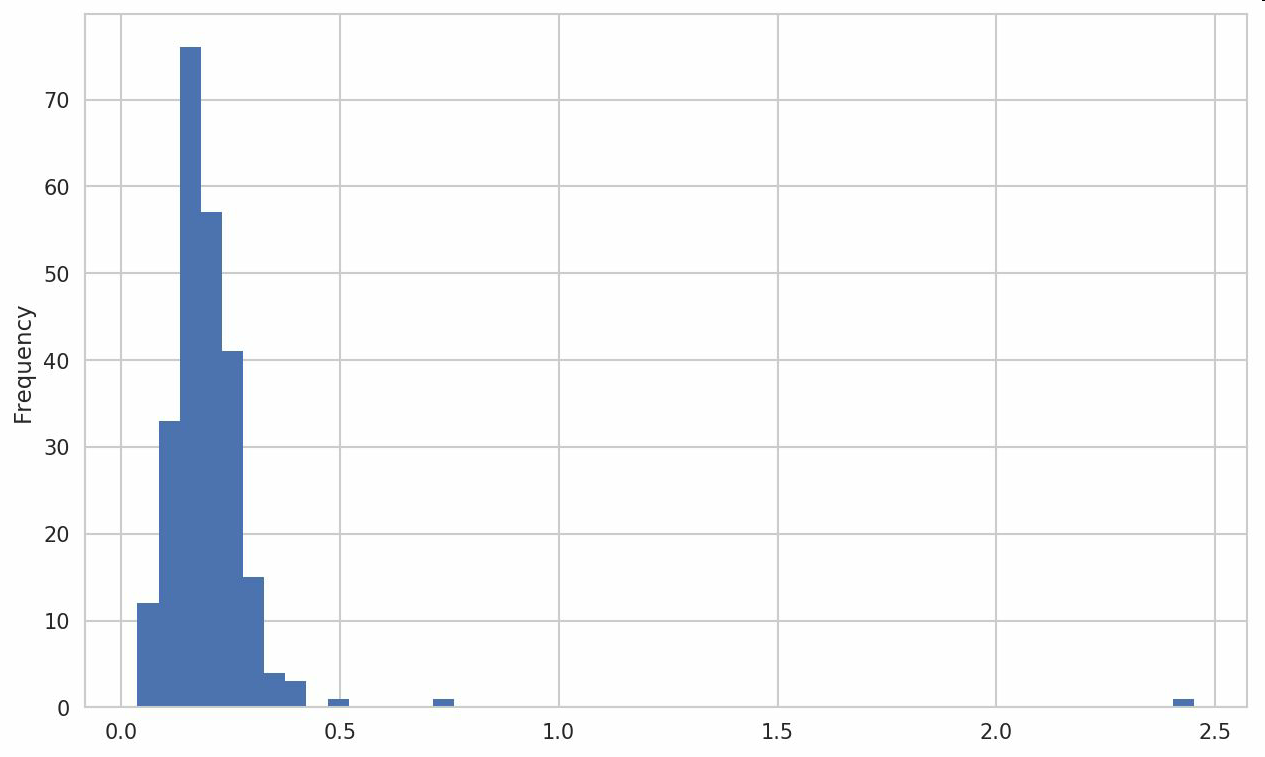In : tips['tip_pct'].plot.density()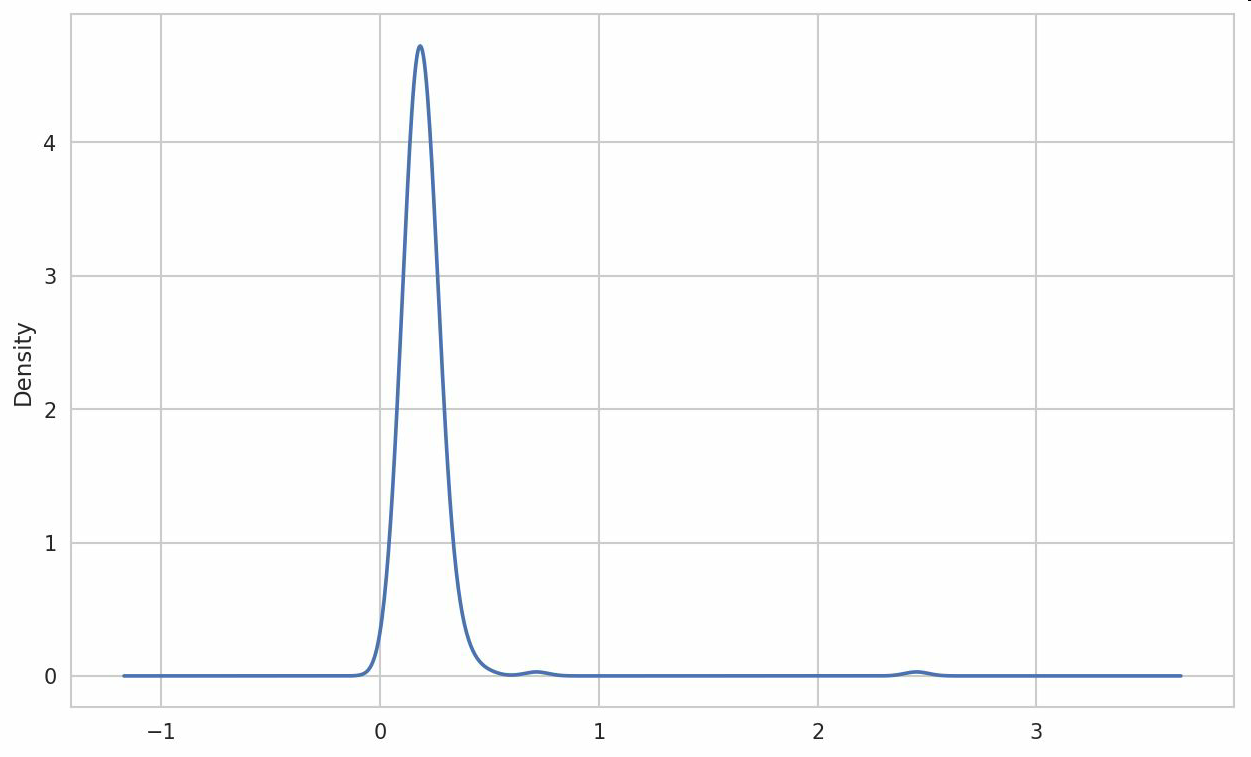seaborn的distplot方法绘制直方图和密度图更加简单，还可以同时画出直方图和连续密度估计图。作为例子，考虑一个双峰分布，由两个不同的标准正态分布组成（见图9-23）：

In : comp1 = np.random.normal(0, 1, size=200)

In : comp2 = np.random.normal(10, 2, size=200)

In : values = pd.Series(np.concatenate([comp1, comp2]))

In : sns.distplot(values, bins=100, color='k')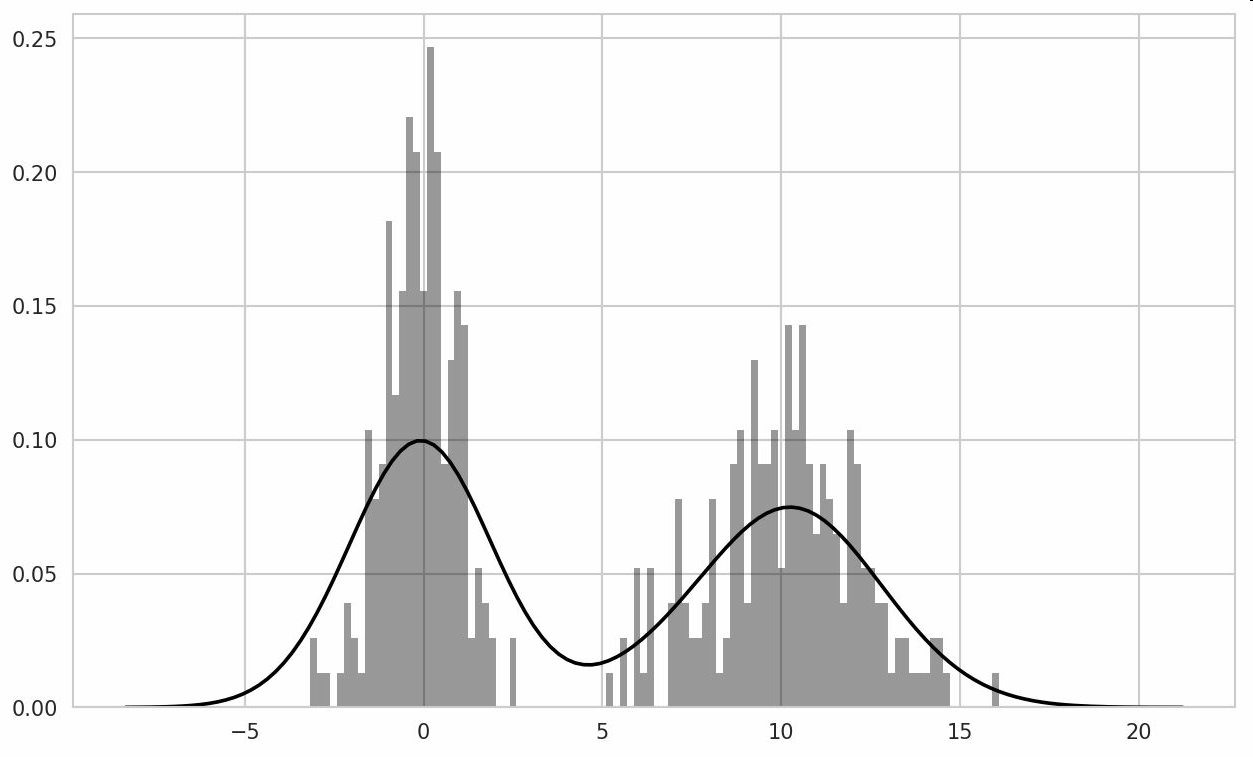## 散布图或点图

In : macro = pd.read_csv('examples/macrodata.csv')

In : data = macro[['cpi', 'm1', 'tbilrate', 'unemp']]

In : trans_data = np.log(data).diff().dropna()

In : trans_data[-5:]
Out:
cpi        m1  tbilrate     unemp
198 -0.007904  0.045361 -0.396881  0.105361
199 -0.021979  0.066753 -2.277267  0.139762
200  0.002340  0.010286  0.606136  0.160343
201  0.008419  0.037461 -0.200671  0.127339
202  0.008894  0.012202 -0.405465  0.042560


In : sns.regplot('m1', 'unemp', data=trans_data)
Out: <matplotlib.axes._subplots.AxesSubplot at 0x7fb613720be0>

In : plt.title('Changes in log %s versus log %s' % ('m1', 'unemp'))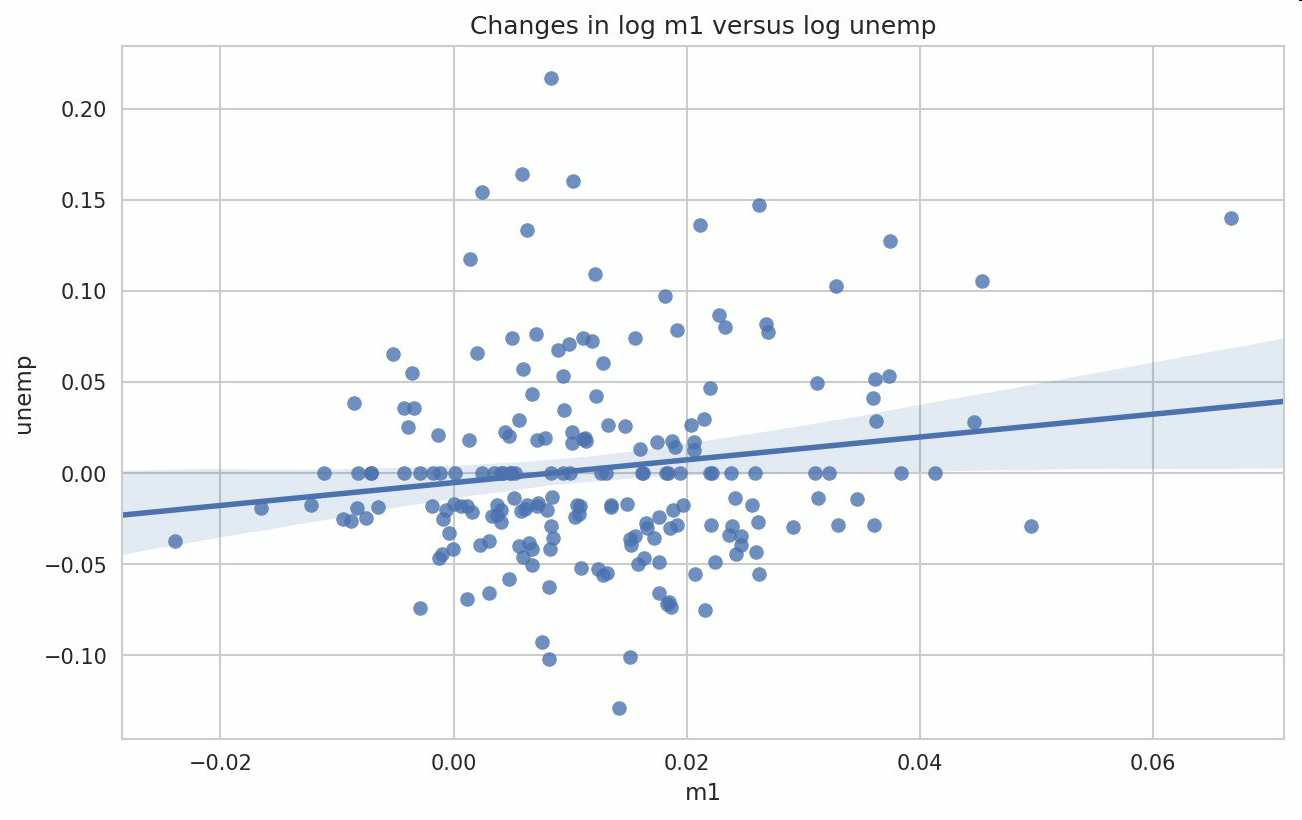In : sns.pairplot(trans_data, diag_kind='kde', plot_kws={'alpha': 0.2})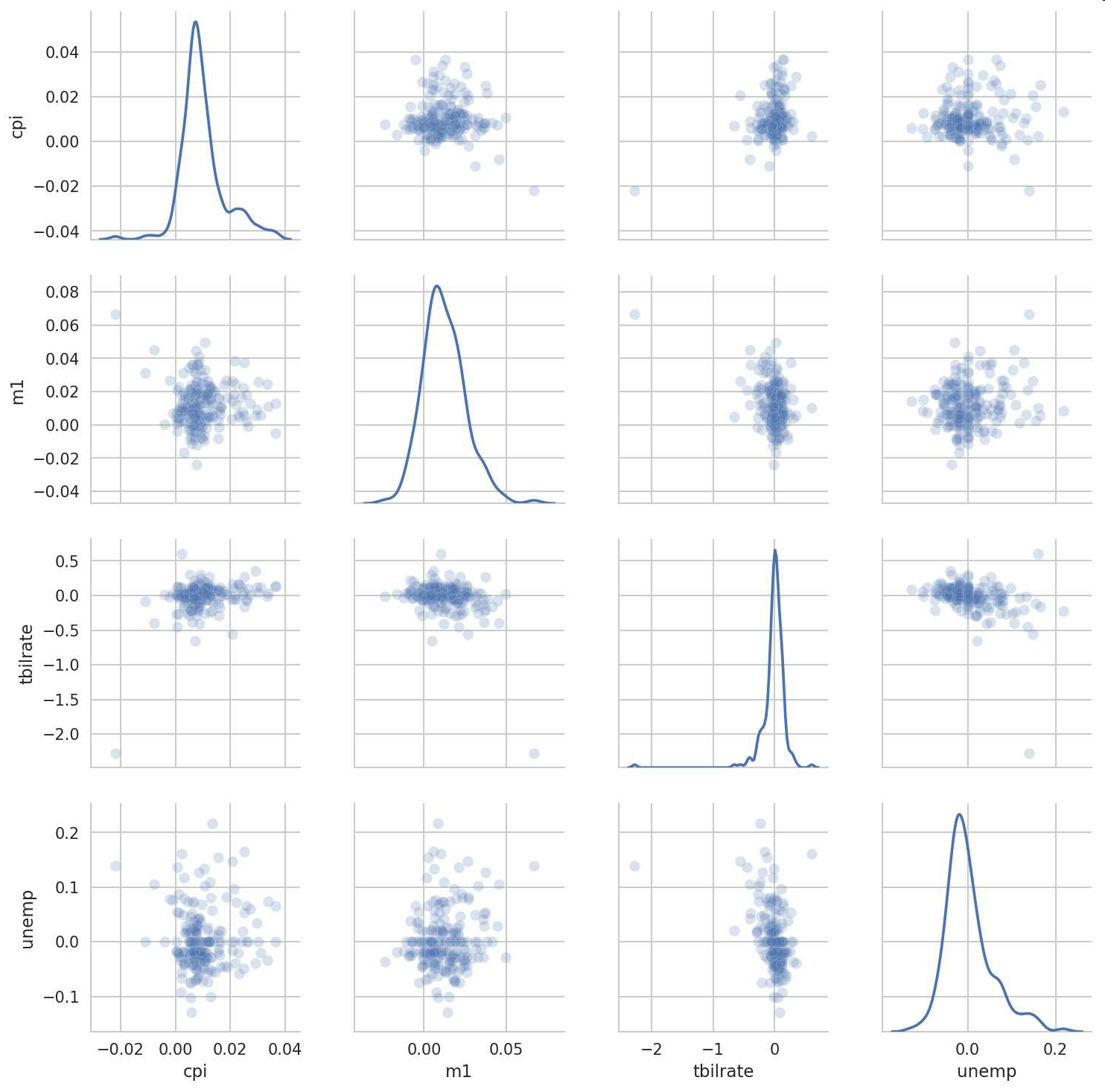## 分面网格（facet grid）和类型数据

 In : sns.factorplot(x='day', y='tip_pct', hue='time', col='smoker',
.....:                kind='bar', data=tips[tips.tip_pct < 1])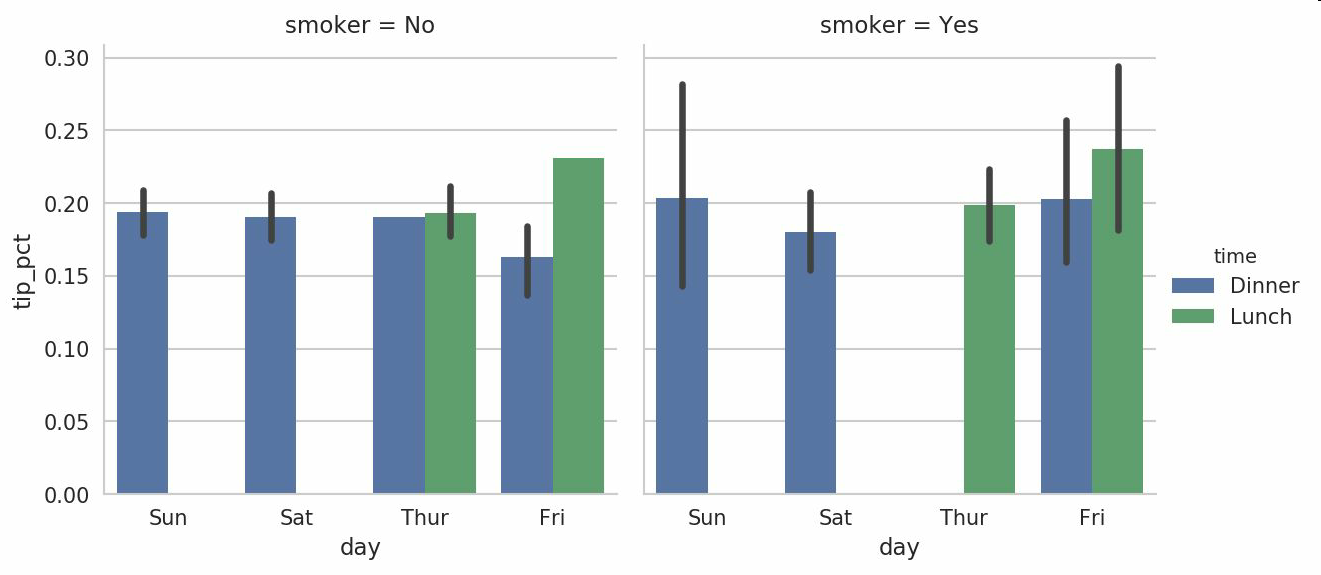In : sns.factorplot(x='day', y='tip_pct', row='time',
.....:                col='smoker',
.....:                kind='bar', data=tips[tips.tip_pct < 1])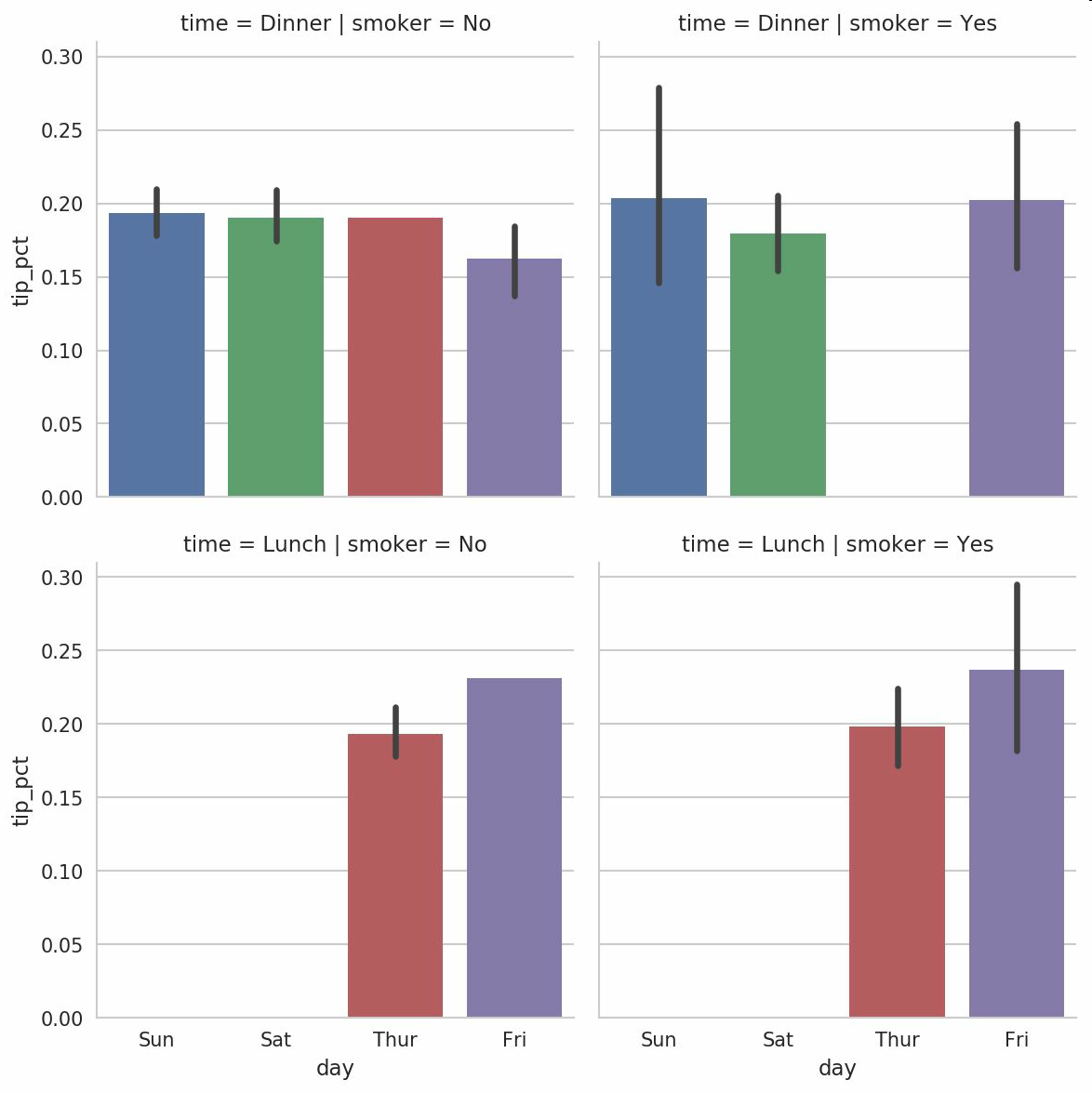factorplot支持其它的绘图类型，你可能会用到。例如，盒图（它可以显示中位数，四分位数，和异常值）就是一个有用的可视化类型（见图9-28）：

In : sns.factorplot(x='tip_pct', y='day', kind='box',
.....:                data=tips[tips.tip_pct < 0.5])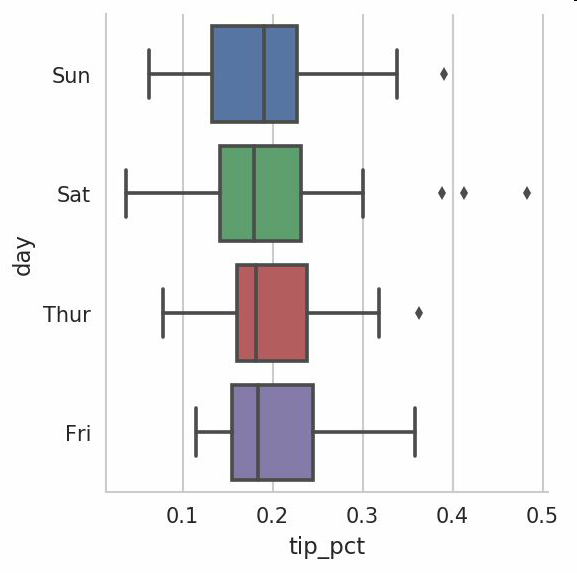# 9.4 总结1207 0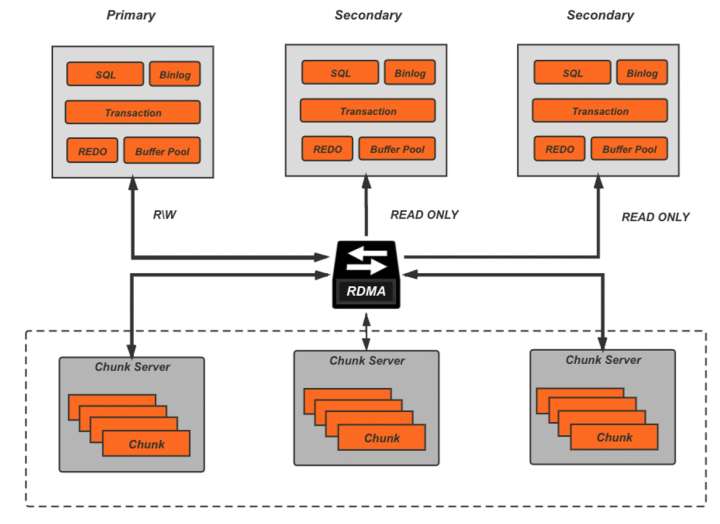PolarDB 并行查询的前世今生

472 0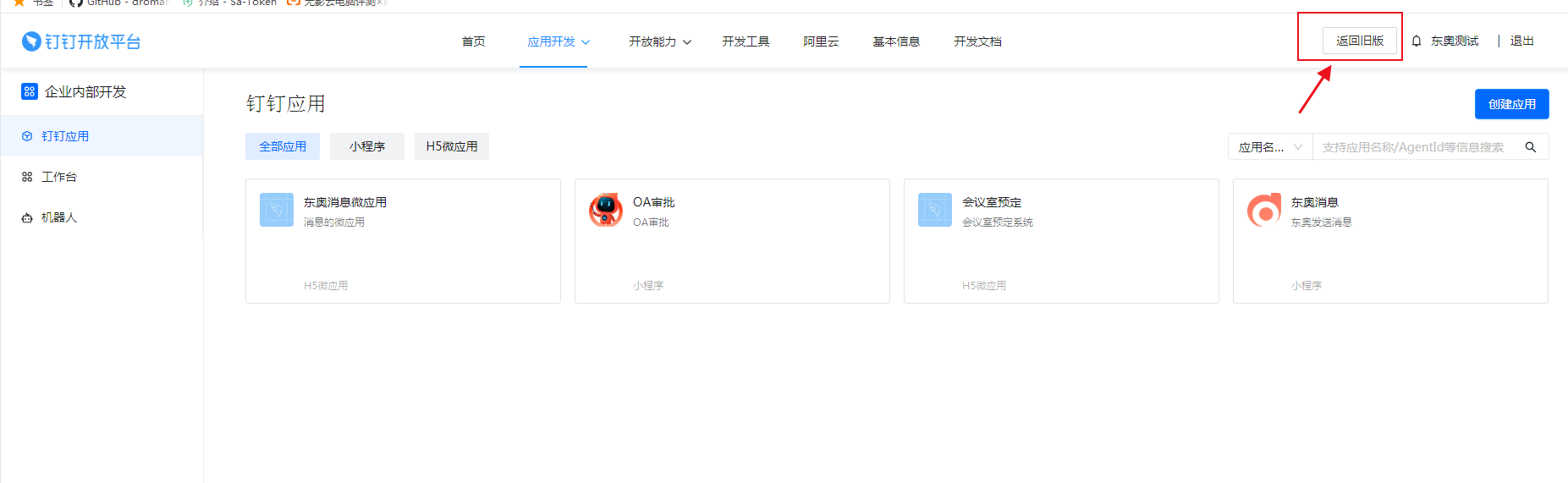1156 0MySQL模糊查询再也用不着 like+% 了！

25663 0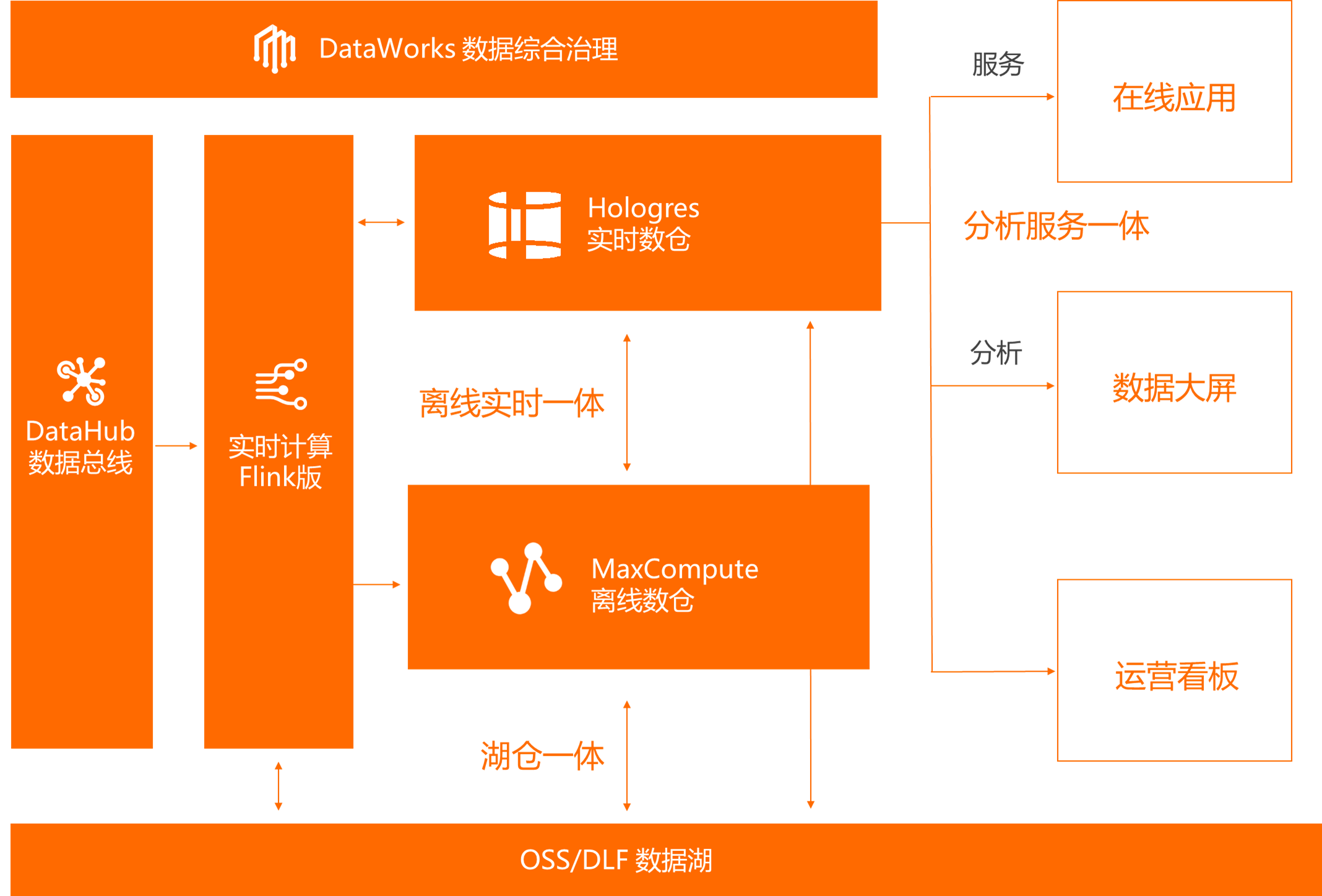1585 0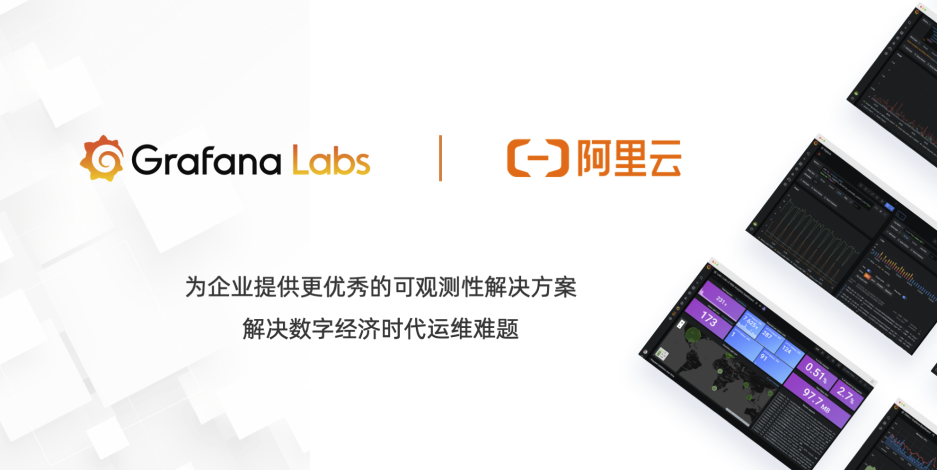Grafana 帮助运维人员轻松处理各类运维过程中遇到的各类数据可视化与分析难题。目前阿里云 Grafana 服务全面免费公测，帮助企业轻松构建运维数据可视化平台，轻松实现数据驱动运维！
1127 0993 0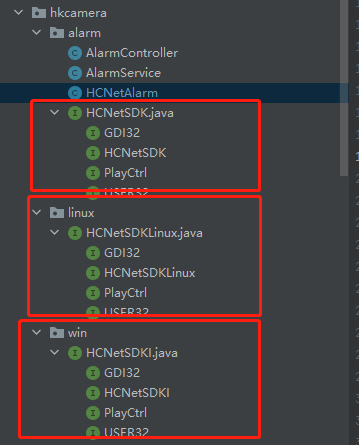springboot-对接海康摄像头，兼容window和Linux环境
1310 01121 0276270 0
seancheney

117

0